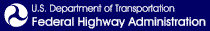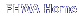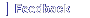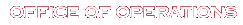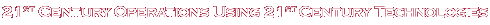# Traffic Analysis Toolbox Volume VI: Definition, Interpretation, and Calculation of Traffic Analysis Tools Measures of Effectiveness

## 4.0 Calculation of MOEs by Traffic Analytical Tools

This chapter presents the results of a comprehensive investigation into how commonly used traffic analytical tools define and calculate commonly used MOEs. First the computation methodologies are explained based upon published user guides for each tool and informal correspondence with the software developers. Then the tools are tested on a simple test bed under both free-flow and congested traffic demand conditions. Variations in the reported MOEs are noted.

### 4.1 Description of MOE Computation Methodologies

This section describes the methodologies used by the operations analysis tools to compute the MOEs that were selected for further investigation in Chapter 2.

#### 4.1.1 HCS

The section describes how the MOEs are computed by the HCS software, Version 5.2, developed by the University of Florida, in 2005.

##### Computation of HCM Level of Service

The HCS software is intended to be a faithful implementation of the Highway Capacity Manual. As such, excepting for programming errors, which are corrected as soon as they are found, the HCS software computes the HCM LOS exactly according to the HCM methods. HCS implements the following HCM methods: Signalized Intersections, Unsignalized Intersections, Urban Streets, Freeway Basic, Freeway Weave, Freeway Ramps, Freeway Facilities, two-lane Rural Roads, Multi-lane Rural Highways, and Transit.

##### Computation of Volume Capacity Ratio

The HCS software computes capacity per the HCM method. [Highway Capacity Manual, Transportation Research Board, Washington D.C., 2000.] The HCM defines capacity as; "The maximum sustainable flow rate at which vehicles or persons reasonably can be expected to traverse a point or uniform segment of a lane or roadway during a specified time period under given roadway, geometric, traffic, environmental, and control conditions…" The HCM capacity for each facility type is computed according to the equations below.

The following equation is used to compute the capacity of all uninterrupted flow facilities (freeways, multi-lane highways, and two-lane rural roads):

Cap = Base Cap * N * fhv * PHF* fp * fg [Equation 3]

Where:

• Cap = Capacity in terms of vehicles per hour.
• Base Cap = Base capacity in terms of passenger cars per hour per lane (varies by facility type).
• N = Number of through lanes.
• fhv = Heavy vehicle adjustment factor (varies by facility type, vehicle mix, and grade).
• PHF = Peak-hour factor (the ratio of the peak 15-minute flow rate to the average hourly flow rate).
• fg = Adjustment factor for grades (used for two-lane highways only).

For freeway and highway weaving sections the capacity is a function of weaving type, number of lanes, free-flows speed, length and weaving ratio. Due to the discontinuous nature of the procedure for weaving sections, no closed form equation for capacity is available.

The following equation is used to compute the lane group capacity for an approach to a signalized intersection:

s = (g/C) so N fw fHV fg fp fbb fa fLU fLT fRT fLpb fRpb [Equation 4]

Where:

• cap = Capacity for subject lane group, expressed as a total for all lanes in lane group (veh/h).
• g/C = Effective green time per cycle ratio for lane group.
• so = Base saturation flow rate per lane (pc/h/ln).
• N = Number of lanes in lane group.
• fw = Adjustment factor for lane width.
• fHV = Adjustment factor for heavy vehicles in traffic stream.
• fp = Adjustment factor for existence of a parking lane and parking activity adjacent to lane group.
• fbb = Adjustment factor for blocking effect of local buses that stop within intersection area.
• fa = Adjustment factor for area type.
• fLU = Adjustment factor for lane utilization.
• fLT = Adjustment factor for left turns in lane group.
• fRT = Adjustment factor for right turns in lane group.
• fLpb = Pedestrian adjustment factor for left-turn movements.
• fRpb = Pedestrian-bicycle adjustment factor for right-turn movements.

The following equation is used to compute the lane group capacity for the approach to a roundabout and for a stop sign controlled approach to an intersection: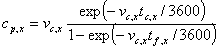[Equation 5]

Equation 5. Lane Group Capacity for Roundabouts and Stop Controlled Intersections. c subscript p, x is equal to the product of v subscript c,x, times the quotient of the exponential function of open parenthesis negative v subscript c,x, times t subscript c,x divided by 3600, close parenthesis; divided by the following: 1 minus the exponential function of open parenthesis (negative v subscript c,x, times t subscript f,x, divided by 3600, close parenthesis.

Where:

• cp,x = Potential capacity of minor movement x (veh/h).
• vc,x = Conflicting flow rate for movement x (veh/h).
• tc,x = Critical gap (s).
• tf,x = Follow-up time for minor movement x (s).

For a roundabout x=1, since there is only one movement on the approach.

The capacity of an all-way stop is a function of the demands on the other approaches and is determined through an iterative procedure.

Capacities and volume/capacity ratios are reported by HCS as follows:

Facility Type

Lane or Movement V/C and Capacity

Overall V/C and Capacity

Signals

Yes – v/c by lane group

Yes – v/c only

Two-Way Stops

Yes – v/c by lane group

No

All-Way Stops

Yes – Capacity by lane group

No

Yes – Upper/lower bounds v/c of approaches

No

Arterial

Yes – Intersection v/c and through capacity

No

Freeway Basic

No

No

Freeway Weave

No

Yes – Capacity only

Freeway Ramp

Yes – Capacity of ramp and two lanes of freeway

No

Freeway System

Yes – Capacity and v/c by freeway segment

No

Two-Lane Highway

Yes – Two-way and one-way v/c

No facility capacity

Multi-Lane Highway

Not applicable

No facility capacity

Transit

No capacity by bus

No capacity by route

##### Computation of Speed and Travel Time

HCS computes mean speeds for urban streets, freeway facilities, multilane highways, and two-lane highways. The HCM formulae used by HCS are explained below.

###### Freeway Facilities

HCS computes the mean speeds for individual freeway segments and time periods using the following procedure from HCM.

If no queuing is present on the segment during any of the time periods then HCS uses the heuristic formula contained in the HCM for basic freeway sections to predict speed given the equivalent passenger volume and the free-flow speed.

If queuing is present on one or more segments during the analysis period then the speed = flow/density equation is used to compute speed: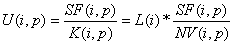[Equation 6]

Equation 6. Average Speed on Freeway Facilities. U open parenthesis i, p, close parenthesis is equal to the quotient of SF, open parenthesis i, p, close parenthesis, divided by K open parenthesis, i, p, close parenthesis, which in turn is equal to a third expression: the product of L, open parenthesis i, close parenthesis, times the quotient of SF, open parenthesis i, p, close parenthesis, divided by NV open parenthesis, i, p, close parenthesis.

Where:

• U(i,p) = The average speed on segment "i" during period "p" (mph).
• SF(i,p) = The segment flow rate (vph).
• K(i,p) = The average segment density (veh/mi).
• L(i) = The length of segment (miles).
• NV(i,p) = The number vehicles.

The segment performance measures are then summed to total and average system performance using the following formulae:[Equation 7]

Equation 7. Space Mean Speed. SMS is equal to the quotient of the summation of p, and summation of i, of the product of SF, open parenthesis i, p, close parenthesis, times L, open parenthesis i, close parenthesis, divided by the following: summation of p, and summation of i, of the product of SF, open parenthesis i, p, close parenthesis, times the quotient of L, open parenthesis i, close parenthesis, divided by U open parenthesis i, p, close parenthesis.

Where:

• K = Mean facility density across all segments (i) and time periods (p) (veh/mi).
• L = Length of segment (miles).
• U = Mean speed on segment (mph).
###### Multilane Highways

For volumes less than capacity a heuristic formula contained in the HCM for multilane highways is used to predict speed given the equivalent passenger volume and the free-flow speed. No procedure is provided for volumes greater than capacity.

###### Two-Lane Highways

For volumes less than capacity HCS uses the following HCM formula for estimating speed for two-lane highways:

ATS = FFS – 0.00776vp  – fnp [Equation 8]

Where:

• ATS = Average travel speed for both directions of travel combined (mi/h).
• FFS = Free-flow speed (mi/h).
• fnp = Adjustment for percentage of no-passing zones.
• vp = Passenger-car equivalent flow rate for peak 15-min period (pc/h).

No procedure is provided for volumes greater than capacity.

###### Urban Streets

HCS uses the following HCM formulae to estimate mean speed (in one direction) for urban streets.[Equation 9]

Equation 9. Space Mean Speed. SMS is equal to the quotient of the product of 3600 times the sum of L subscript i, divided by the sum of the product of RT times sum of i for L subscript i, plus sum of j for d subscript j.

Where:

• SMS = Space mean speed over the length of the facility (mph or kph).
• Li = Length of segment "i" (mph or kph).
• RT = Running time per mile (sec/mile or sec/km).
• dj = Delay at signal "j" for through traffic in the subject direction.

The delay for through traffic at each signal is computed according to the following formulae.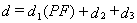[Equation 10]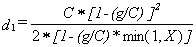[Equation 11]

Equation 11. d subscript 1 equals the quotient of the product of C times the square of the difference of 1 minus the quotient of g divided by C, divided by the product of 2 times the difference of 1 minus the product of the quotient of g divided by C times the minimum of either 1 or X.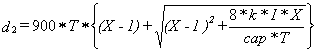[Equation 12]

Equation 12. d subscript 2 equals the product of 900 times T times the sum of the difference of X minus 1, plus the square root of the following: the sum of the square of X minus 1 plus the quotient of the product of 8 times k times I times X divided by the product of cap times T.

Where:

• d = Control delay (sec/veh).
• d1 = Uniform delay (sec/veh).
• d2 = Incremental delay (sec/veh).
• d3 = Initial queue delay (sec/veh).
• PF = Progression adjustment factor.
• C = Cycle length (secs).
• g = Effective green time for the lane group (secs).
• X = Volume/capacity ratio for the subject lane group.
• k = Actuated control factor.
• I = Upstream filtering factor.
• v = Volume per hour (veh/hr).
• cap = Capacity (sat * g/c) for the through lane group (veh/hr).
• T = Duration of analysis period (hr).
##### Computation of Delay

HCS computes delay only for intersections. The analyst must use the output speeds for freeway facilities, multilane highways, two-lane highways, and urban streets to manually compute facility delay.

###### Freeway Facilities

The HCM does not provide a procedure for estimating delay for freeway facilities, so the HCS does not output this MOE. However the analyst can manually compute facility delay by dividing the predicted speed into the length of the facility to get travel time. Subtracting the predicted travel time from the free-flow travel time gives facility delay.

###### Multilane Highways

The HCM does not provide a procedure for estimating delay for multilane highways, so the HCS does not output this MOE. It can be estimated manually by the analyst from the HCS outputs as described above for freeway facilities.

###### Two-Lane Highways

The HCM does not provide a procedure for estimating delay for two-lane highways, so the HCS does not output this MOE. It can be estimated manually by the analyst from the HCS outputs as described above for freeway facilities.

###### Urban Streets

Urban street speed is computed by HCS but not delay. Mean and total facility delay can be estimated manually by the analyst using the HCS outputs as described above for freeway facilities.

The HCS does directly compute delay for each individual signalized intersection as described above under speed estimation for urban streets.

Unsignalized intersection delay is computed by HCS using an intersection approach delay formula similar to the one described above for signalized intersections.

##### Computation of Queue
###### Freeway Facilities

HCS computes queue lengths for freeway facilities based on an assumed linear relationship between flow and density. The density at capacity is determined based upon the capacity flow rate and the mean speed at capacity. The capacity and the speed at capacity are taken from the basic freeway section speed-flow curves in the HCM. They vary according to the free-flow speed for the freeway.

Once the density at capacity is known, then the flow rate is assumed to decrease linearly until it reaches zero at an assumed jam density of 190 passenger cars per lane-mile.

The queue density is computed as follows: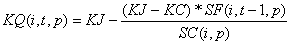[Equation 13]

Equation 13. Queue Density. KG open parenthesis i,t,p close parenthesis is equal to the difference of KJ minus the quotient of the product of the difference of KJ minus KC times SF open parenthesis i, t minus 1, p close parenthesis, divided by SC open parenthesis i,p close parenthesis.

Where:

• KQ(i,t,p) = The density of queue on segment "i," at time step "t" for period "p" (veh/mi).
• KJ = The jam density (veh/lane/mi).
• KC = The density at capacity (veh/lane/mi).
• SF(1,t-1,p) = The segment flow (veh/hr).
• SC(i,p) = The segment capacity (veh/hr).

The number of vehicles in the queue divided by the queue density and then divided by the number of lanes gives the length of the queue.

###### Multilane Highways

The HCM does not provide a procedure for estimating queues for multilane highways, so the HCS does not output this MOE.

###### Two-Lane Highways

The HCM does not provide a procedure for estimating queues for two-lane highways, so the HCS does not output this MOE.

###### Urban Streets

The HCM does not compute queuing directly for urban streets, so HCS does not do so either. The HCM and HCS do compute queues for intersections.

The mean queue for a lane group approaching a signalized intersection is computed according to the following formulae.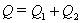[Equation 14]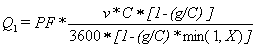[Equation 15]

Equation 15. Q subscript 1 equals the product of PF times the quotient of the product of v times C times the difference of 1 minus the quotient of g divided by C, divided by the product of 3600 times the difference of 1 minus the product of the quotient of g divided by C times the minimum of either 1 or X.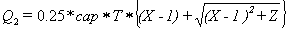[Equation 16]

Equation 16. Q subscript 2 equals the product of 0.25 times cap times T times the sum of the difference of X minus 1 plus the square root of the following: the sum of the square of X minus 1 plus Z.

Where:

• Q = Control delay (sec/veh).
• Q1 = Uniform delay (sec/veh).
• Q2 = Incremental delay (sec/veh).
• PF = Progression adjustment factor.
• C = Cycle length (secs).
• g = Effective green time for the lane group (secs).
• X = Volume/capacity ratio for the subject lane group.
• Z = Composite factor (see page 16-153 of HCM for details).
• v = Volume per hour (veh/hr).
• cap = Capacity (sat * g/c) for the through lane group (veh/hr).
• T = Duration of analysis period (hr).

The HCM also provides for calculating various percentile probability queues as a function of the mean queue.

##### Computation of Stops

The HCM does not provide any procedures for computing number of stops. Thus HCS does not provide this MOE.

##### Computation of Density
###### Freeway Facilities

If no queuing is present the equivalent passenger car flow rate per lane is divided by the mean speed to obtain the density in units of equivalent passenger cars per lane-mile. If queuing is present then the following equation is used: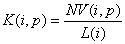[Equation 17]

Equation 17. K open parenthesis i,p close parenthesis equals the quotient of NV open parenthesis i,p close parenthesis, divided by L open parenthesis, i, close parenthesis.

Where:

• K(i,p) = The average segment density (veh/mi).
• L(i) = The length of segment (miles).
• NV(i,p) = The number vehicles.

The segment performance measures are then summed to total and average system performance using the following formula:[Equation 18]

Equation 18. K equals the quotient of the summation over p, summation over i of the product of K open parenthesis i,p closer parenthesis times L open parenthesis i close parenthesis, divided by the summation over p, summation over i of the product of L open parenthesis i close parenthesis times NV open parenthesis i,p closer parenthesis.

Where:

• K = Mean facility density across all segments (i) and time periods (p) (veh/mi).
• L = Length of segment (miles).
• U = Mean speed on segment (mph).
• NV = Number of vehicles on segment.
###### Multilane Highways

HCS computes the mean passenger car density by dividing the mean speed into the passenger car equivalent flow rate.

###### Two-Lane Highways

The HCM does not provide a procedure for estimating delay for two-lane highways, so the HCS does not output this MOE. However the analyst could divide the mean speed into the flow rate to obtain density if desired.

###### Urban Streets

The HCM does not provide a procedure for computing density on urban streets and therefore HCS does not output this MOE for urban streets.

##### Computation of Travel-Time Variance

The HCM does not provide any procedures for any facilities for computing travel-time variance. Thus HCS does not provide this MOE.

#### 4.1.2 Synchro 6

This section describes the formulae used by Synchro 6 (Build 614), released 2005 by Trafficware of Sugarland, Texas. [Source: Synchro6 Help.] Synchro is designed to evaluate the performance of urban streets, signalized intersections, and unsignalized intersections (two-way stop, all-way stop, and single-lane roundabouts). Synchro does not provide performance data for freeways, multilane highways, or two-lane rural roads.

##### Computation of HCM Level of Service

Synchro computes the signalized intersection, unsignalized intersection, and arterial LOS according to the HCM. For signalized intersections, Synchro provides the user with two additional intersection levels of service (LOS). One is called the Intersection LOS; the other is called the ICU LOS.

The Intersection LOS is based upon a "percentile" control delay computation but uses the same numerical delay thresholds as the HCM. The percentile control delay is different than the HCM control delay for the intersection. The computation is explained below under the delay MOE.

The ICU LOS is based upon the critical flow ratio (ICU) for the intersection. The critical flow ratio is the sum of green +clearance times required to serve the critical movements at the intersection divided by a reference cycle length. The ICU LOS is designed to be insensitive to the actual signal timing for the intersection.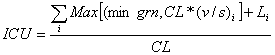[Equation 19]

Equation 19. ICU equals the quotient of the summation over i of the sum of the maximum of either min grn or the product of CL times open parenthesis, ratio of v to s, close parenthesis subscript i plus L subscript i divided by CL.

Where:

• ICU = Intersection capacity utilization ratio.
• Min grn = Minimum green time for critical movement "i" (secs).
• CL = Reference cycle length (secs). Set to 120 seconds.
• L = Loss time for critical movement "i" (secs).

The ICU is converted into a letter LOS grade according to Table 13.

Table 13. ICU LOS Thresholds

LOS

Maximum ICU

A

55%

B

64%

C

73%

D

82%

E

91%

F

100%

G

109%

H

over 109%

##### Computation of Volume Capacity Ratio

Synchro provides volume/capacity ratios calculated using HCM saturation flow rates for signalized intersections, and unsignalized intersections (including two-way stop, all-way stop, and roundabouts).

Synchro provides volume/capacity ratios at intersections for each lane group. Capacity is computed using the HCM saturation flow computation method.

For the intersection as a whole, Synchro reports the maximum lane group v/c ratio for the intersection. A special HCM report provides the critical v/c (x) that is computed according to the HCM.

##### Computation of Speed and Travel Time

Synchro computes the arterial travel time according to the HCM method.

##### Computation of Delay

Synchro computes control delay according to the HCM method for signalized intersections, two-way stops, and all-way stops. Control delay is not computed for roundabouts in accordance with the HCM.

For signalized intersections Synchro also provides an alternative computation of delay based upon an assumed distribution of traffic volumes for the intersection. This is called the Percentile Delay to distinguish it from the HCM control delay.

The percentile delay computation uses only the first and third delay terms of the HCM. The incremental delay and the progression factor for the uniform delay in the HCM method are dropped. The incremental delay term of the HCM is modified for X>1 to use the saturation flow rate. The fourth term, queue delay, is added to cover delays due queue blockages resulting in unused green time.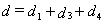[Equation 20]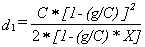if X<= 1.00 [Equation 21]

Equation 21. d subscript 1 equals the quotient of the product of C times the square of the difference of 1 minus the quotient of g divided by C, divided by the product of 2 times the difference of 1 minus the product of the quotient of g divided by C, times X, if X is less than or equal to 1.00.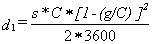if X> 1.00 [Equation 22]

Equation 22. d subscript 1 equals the quotient of the product of s times C times the square of the difference of 1 minus the quotient of g divided by C, divided by the product of 2 times 3600, if X is greater than 1.00.

Where:

• D = Delay (sec/veh).
• d1 = Uniform delay (sec/veh).
• d3 = Initial queue delay (sec/veh).
• d4 = Queue interaction term (sec/veh).
• C = Cycle length (secs).
• S = Saturation flow rate (veh/hr-green).
• G = Effective green time for the lane group (secs).
• X = Volume/capacity ratio for the subject lane group.

Synchro introduces the random component of delay (that, in the HCM, was contained in the incremental delay term) by computing the uniform delay term using a series of 5 volume scenarios. Uniform delay (d1) is computed for the 90th percentile volume, the 70th percentile volume, the 50th percentile volume, the 30th percentile volume, and the 10th percentile volume. The 5 delay estimates are averaged to obtain the average delay.

If the traffic signal is traffic actuated, then the green times also are computed for each percentile volume when computing the percentile delay.

The percentile volumes are computed assuming a Poisson arrival pattern with mean equal to the input traffic volume for the lane group divided by the number of cycles per hour.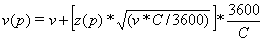[Equation 23]

Equation 23. V open parenthesis, p, close parenthesis equals the sum of v plus the product of the quotient of 3600 divided by C times z open parenthesis, p, close parenthesis times the square root of the following: the product of v times the quotient of C divided by 3600.

Where:

• v(p) = Percentile volume for percentile "p" (veh/hr).
• V = Lane group volume (veh/hr).
• z(p) = Number of standard deviations for percentile "p" for Poisson Distribution.
• z(p) = -1.28 for 10%.
• z(p) = -0.52 for 30%.
• z(p) = 0 for 50%.
• z(p) = +0.52 for 70%.
• z(p) = +1.28 for 90%.
• C = Cycle length (secs).

The initial queue delay (d3) is the same as the HCM term.

The queue interaction term (d4) takes into the account blockages and demand starvation resulting from queues that extend longer than the available distance between intersections.

If Min[CapDist, VolDist) > Distance, [Equation 24]

Then queue interaction is likely to occur.

Where:

• Distance = Available distance between intersections to store queues (ft).
• CapDist = Distance required to store a flow at capacity.
• CapDist = AVL * [2+g*s/(n*3,600)].
• VolDist = Distance required to store the 90% volume.
• VolDist = AVL* [2+v90*C/n*3,600)].
• AVL = Average Vehicle Length (ft).
• G = Green time per cycle (secs).
• S = Saturation flow rate (veh/hr-green).
• N = Number of lanes.
• v90 = 90th percentile volume.
• C = Cycle length (secs).

If the queue interaction is likely to occur then Synchro calculates the likely reduction in capacity flow rates at the downstream intersection for the 50 percentile and 90 percentile volumes. The queue interaction delay is then computed using the HCM incremental delay formula (d2) and the reduced capacities.

The storage blocking portion of the queue interaction calculation was recently disabled (as of Build 610) due to problems with its representation of short left turn pocket blockages.

##### Computation of Queue

For unsignalized intersections Synchro computes queue lengths according to the HCM method.

For signalized intersections Synchro computes queues for the 50 percentile and 95 percentile volumes. The 50 percentile queue is computed as follows.

If the volume is less than capacity then the following formula is used.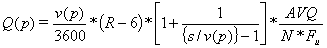[Equation 25]

Equation 25. Q open parenthesis, p, close parenthesis equals the product of the quotient of v open parenthesis, p, close parenthesis divided by 3600, times the difference of R minus 6, times the sum of 1 plus the quotient of 1 divided by the difference of the quotient of s divided by v open parenthesis, p, close parenthesis minus 1, times the quotient of AVQ divided by the product of N times F subscript u.

If the volume is equal to or greater than capacity then the following formula is used.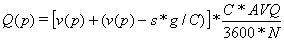[Equation 26]

Equation 26. Q open parenthesis, p, close parenthesis equals the product of the sum of v open parenthesis, p, close parenthesis plus the difference of v open parenthesis, p, close parenthesis minus the product of s times the quotient of g divided by C, times the quotient of the product of C times AVQ divided by the product of 3600 times N.

Where:

• Q(p) = Queue length for percentile "p" volume (ft).
• v(p) = Percentile "p" volume for lane group (veh/hr).
• R = Red time (secs).
• S = Saturation flow rate (veh/hr-green).
• AVQ = Average front-to-front spacing of vehicles in queue (ft).
• N = Number of lanes in lane group.
• Fu = Lane utilization factor.
• C = Cycle length (secs).
• PHF = Peak-hour factor.

A special value of the 95 percentile volume is used to compute the 95 percentile queue.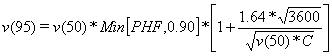[Equation 27]

Equation 27. v open parenthesis, 95, close parenthesis equals the product of v open parenthesis, 50, close parenthesis, times the minimum of either PHF or 0.90, times the sum of 1 plus the quotient of the product of 1.64 times the square root of 3600 divided by the square root of the product of v open parenthesis, 50, close parenthesis times C.

If the upstream signal has the same cycle length as the subject intersection, then the 95 percentile volume on the subject approach is compared to the maximum volume that can be delivered by the upstream signal and reduced accordingly for the "metering" effect of the upstream signal.

##### Computation of Stops

Synchro computes the number of stops for a signalized intersection approach as equal to the number of vehicles queued multiplied by a stopping rate percentage obtained from Transyt 7-F (see Table 14).

Table 14. Conversion of Delay to Stops

Delay (Secs)

Percent Stopping

0

0%

1

20%

2

58%

3

67%

4

77%

5

84%

6

91%

7

94%

8

97%

9

99%

10 secs or greater

100%

##### Computation of Density

Synchro does not compute density.

##### Computation of Travel-Time Variance

Synchro does not compute travel-time variance.

#### 4.1.3 Dynasmart-P

This section discusses Dynasmart-P Version 1.0, August 2004. [Hani S. Mahmassani, Hayssam Sbayti, and Xuesong Zhou, Dynasmart-P Version 1.0 User's Guide, Federal Highway Administration, Office of Operations Research and Development, McLean, Virginia, August 2004.]

##### Overview of MOEs

Dynasmart-P outputs overall vehicle statistics for the network, including trip times, travel times, stop times, entry queues, and travel distances. Also, the following link-specific statistics are provided averaged over all simulation intervals.

1. Number of generated vehicles;
2. Number of vehicles;
3. Vehicle queue length (number of vehicles in queue);
6. Mean Speed of moving vehicles; and
7. Mean PC Density of moving vehicles.

Dynasmart also can output individual vehicle trajectories enabling the user to use ACCESS or to write a program to compute their own custom measures of effectiveness.

##### Computation of HCM Level of Service

Dynasmart-P does not output HCM level of service.

##### Computation of Volume Capacity Ratio

Dynasmart-P does not output link volume/capacity ratios. However, the analyst can compute the v/c ratios by dividing the output link volumes by the input link capacities.

##### Computation of Speed and Travel Time

Dynasmart-P outputs system total trip distance (VMT), trip time (VHT), network entry delay (entry queue time), stop time, and average speed. Dynasmart-P also outputs link-specific mean speeds and mean speeds outside of queues.

The total trip distance is the sum of the vehicle volume on each link multiplied by the link length. Note that Dynasmart requires that all links be longer than the distance that can be traveled in one simulation interval (six seconds) at the free-flow speed. The software will increase the coded link length as necessary to meet this requirement. Thus some minor distortions of the distance traveled may occur for shorter links that fail to meet this requirement.

The total trip time is the sum of the vehicle volume on each link divided by the mean link speed plus the entry delay time for vehicles denied entry to the network.

The system average speed is the system distance traveled divided by the system trip time (inclusive of delays to vehicles denied entry to the network).

The link-specific mean speed is the simple arithmetic average of the spot speeds of all vehicles on the link at the end of each six-second simulation interval. The user can average the spot speeds over multiple simulation intervals to obtain an hourly average for each link. The variance of the mean speeds can be computed manually by reporting the mean speed for each simulation interval and porting the file into a spreadsheet.

The mean speed of vehicles on a link is estimated by Dynasmart-P using a modified Greenshields equation. A two-regime equation is used for freeways (shown below). A single regime equation is used for arterials.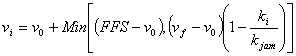[Equation 28]

Equation 28. v subscript i equals the sum of v subscript 0 plus the minimum of either the difference of FFS minus v subscript 0, or the product of the difference of v subscript f minus v subscript 0 times 1 minus the quotient of k subscript I divided by k subscript jam.

Where:

• Vi = Mean speed (mph).
• V0 = Minimum mean speed (mph).
• FFS = Free-flow speed (mph).
• Vf = Hypothetical speed at y-intercept of Greenshields equation.
• Ki = Mean density (pc/lane-mile).
• Kjam = Theoretical jam density (pc/lane-mile).

The single regime equation uses the same equation above with the hypothetical y-intercept speed (vf) set equal to the actual free-flow speed (FFS).

##### Computation of Delay

Dynasmart-P does not output delay statistics, however; the analyst can compute the delay for individual links using the link data output by Dynasmart-P. The individual link delays can be summed to system totals in a spreadsheet.

##### Computation of Queue

The method used by Dynasmart-P to identify queued vehicles on the network is not described in the Users Guide. [This is not documented in User Guide. Response from the developer regarding their definition of queues not received in time for publication.]

##### Computation of Stops

Dynasmart-P tallies stop time for vehicles at a complete stop (zero speed).

##### Computation of Density

Dynasmart-P reports the average equivalent passenger car density by link (pc/mile/lane) averaged over as many six-second simulation intervals as desired by the analyst. A separate density also can be reported for the queue-free portion of each link.

Trucks are converted to passenger car equivalents using the PCE values for each link input by the analyst.

The extra storage space available on links with left and/or right turn bays is not included in the denominator of the density computation. However vehicles in these bays are included in the numerator.

The impacts of partial lane closures on the available storage space due to work zones and incidents are not included in the density computation.

Note that the density computation may be distorted slightly for short links with lengths less than FFS*528/60 (where FFS is the free-flow speed). Dynasmart-P will increase the lengths of the short links to equal the minimum length.

##### Computation of Travel-Time Variance

Dynasmart-P does not output variance statistics. Vehicle trajectories are output by Dynasmart-P which would enable an analyst working with database or similar high-capacity data processing software to compute variances.

However, the finest level of disaggregation available in Dynasmart is the link and six-second-long simulation intervals. Acceleration or deceleration within the link are not treated. Variation of vehicle speeds around the link mean speed is not included in the model. Thus the variances computed from the vehicle trajectories would not be expected to be as large as would be observed in the field.

#### 4.1.4 SIMTRAFFIC

This section describes the formulae used by SimTraffic 6 (Build 614), released 2005 by Trafficware of Sugarland, Texas. [Source: SimTraffic 6 Help.] SimTraffic is based on CORSIM. As such, it is designed to evaluate the performance of freeways, urban streets, signalized intersections, and unsignalized intersections (two-way stop, all-way stop, and single-lane roundabouts). SimTraffic does not model passing in opposing flow lanes, and thus is not designed to evaluate two-lane rural roads.

##### Computation of HCM Level of Service

SimTraffic does not output levels of service.

##### Computation of Volume Capacity Ratio

SimTraffic does not compute capacity or volume/capacity ratios.

##### Computation of Speed and Travel Time

SimTraffic outputs travel time and average speed by turn movement, for arterials, and for the entire network.

The Travel Time (VHT) is the total time each vehicle was present on the link. The travel time includes time spent by vehicles Denied Entry (waiting to enter the network), but does not include the spent by vehicles on the upstream link waiting to enter the subject link.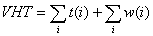[Equation 29]

Where:

• VHT = Total vehicle hours traveled on link or spent in zone waiting to access link (hr).
• t(i) = Time spent by vehicle "i" on link (hr).
• w(i) = Time spent by vehicle "i" in zone waiting to enter link (hr).

The Average Speed is calculated by dividing Total Distance (VMT) by Total Time spent on the network. The time used in the calculation of Average Speed does not include time spent by denied entry vehicles while they are waiting to enter the network. Average speed may therefore be higher than Total Distance (VMT) divided by Total Time (VHT) for the link.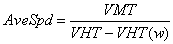[Equation 30]

Where:

• AveSpd = Average link speed (mph).
• VMT = Total vehicle miles traveled on link (mi).
• VHT = Total vehicle hours traveled on link or spent in zone waiting to access link.
• VHT(w) = Total vehicle hours spent by vehicles in zone waiting to access link.

The Total Distance (VMT) is the total distance traveled by all vehicles on the link. Vehicles waiting to enter the link do not travel any distance on the link, so they have no impact on the VMT computation.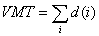[Equation 31]

Where:

• VMT = Total vehicle miles traveled on link (mi).
• d(i) = Distance traveled by vehicle "i" on link (mi).

The Arterial Report is a special report that reports delay, travel time (VHT), distance (VMT), and speed for a select subset of vehicles on a select subset of links. It uses origin destination data to only count vehicles on the current link that came from the arterial on the next upstream link. This is not the same as taking the travel time of only those vehicles that travel the entire length of the arterial.

##### Computation of Delay

SimTraffic outputs total delay, delay/vehicle, and stopped delay by turn movement, for arterials, and for the entire network.

Total Delay is defined as the travel time (VHT) minus the time it would take the vehicle if traveling at the maximum permitted speed (the speed limit or the maximum safe turning speed, whichever is lesser). The delay accrued by vehicles denied entry to the network during each 1/10 second time slice is added to this total.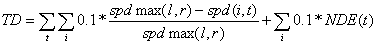[Equation 32]

Equation 32. TD equals the sum of summation over t , summation over i of the product of 0.1 times the quotient of the difference of spdmax open parenthesis, l,r, close parenthesis minus spd open parenthesis, i,t, close parenthesis divided by spdmax open parenthesis, l, r, close parenthesis, plus the summation over i of the product of 0.1 times NDE open parenthesis, t, close parenthesis.

Where:

• TD = Total vehicle-delay (hr).
• spdmax = Maximum speed on link "l" for turn "r."
• spdmax = Link speed limit except when approaching turn, during turn, and accelerating out of turn.
• spd = Actual vehicle speed for vehicle "i" at time slice "t." Each time slice = 1/10 of second.
• NDE = Number of vehicles denied entry during time slice "t."

Delay per Vehicle is the total delay divided by the Number of Vehicles.

The Number of Vehicles is defined as the number of vehicles that exited the link during time slice "t": plus one-half of those vehicles remaining on the link at the end of time slice "t" minus one-half of those vehicles that were present anywhere on the link at the start of time slice "t." The assumption is that vehicles that were present at the start of the time slice and vehicles that were present at the end of the time slice contributed delay for half the time as for vehicles both entering and exiting the link during the time slice.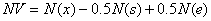[Equation 33]

Where:

• NV = Number of vehicles.
• N(x) = Number of vehicles exiting the link during time period.
• N(s) = Number of vehicles present on link at start of time period.
• N(e) = Number of vehicles present on link at end of time period.
• Spd = Actual vehicle speed for vehicle "i" at time slice "t." Each time slice = 1/10 of second.
• NDE = Number of vehicles denied entry during time slice "t."

Note that this formula results in zero vehicles if the link is totally jammed with vehicles during the time period with nobody entering or exiting during the time period.

The Stopped Delay is the sum over all time slices of the number of vehicles traveling at less than 10 ft/s (3 m/s) times the length of the time slices (1/10 of one second). The sum of the number of denied entry vehicles each time slice times the length of the time slices is added to the stopped delay.

Stop Delay/Vehicles is equal to the Stopped Delay divided by the Number of Vehicles.

##### Computation of Queue

SimTraffic outputs Maximum queue (ft), Average queue (ft), and 95 Percentile queue (feet) by lane by turn movement. SimTraffic also outputs the Upstream Block Time, Storage Block Time, and the queuing penalty.

The maximum queue may be less than the 95 percentile queue because the Maximum queue is observed during the simulation run and the 95 percentile queue is statistically estimated from the mean queue and the standard deviation.

A vehicle is considered queued when it is traveling at less than 10 ft/s (3 m/s) and either at a stop bar or following another queued vehicle. Thus, single vehicle queues are not possible except at intersection stop bars.

All queues in SimTraffic originate at the intersection. Queues cannot originate mid-link.

For queue length computations SimTraffic uses a front-of-vehicle to front-of-vehicle length of 19.5 feet (6 meters).

The Maximum Queue (feet) is the maximum observed distance from the stop bar to the back of queue over the analysis period. Note that Maximum Queue is limited by the length of the link.

Maximum queues are recorded every two minutes during the simulation.

The Average Queue is the average of the observed maximum queues for each of those two-minute periods.

The standard deviation of the maximum queue is computed for all of the two-minute maximum queues recorded during the simulation.

The 95 Percentile queue is computed as 1.65 standard deviations above the average queue.

Thus the 95 Percentile Queue can be greater than the Maximum Queue. Also, the computed 95 Percentile Queue is NOT limited by the length of the link.

SimTraffic will exclude from the queuing statistics most vehicles that stop for a lane change (depending on the circumstances).

The Upstream Block Time is the percent of time that the upstream end of the lane in a link is blocked.

The Queuing Penalty is the traffic volume in the lane times the percent of time during the simulation period that the lane is blocked. For a storage bay the turning volume in the adjacent through lane may be added to the Queuing Penalty volume if the through lane back up is preventing vehicles from accessing the turn bay.

The Storage Bay Block Time is the sum of the percent of time that the storage bay is full and the percent of time that queues in the adjacent through lane exceed the length of the turn bay.

Links may have bend nodes in them. The queue for the intersection at the end of the link includes the queue for the portion of the link upstream of the bend node. A separate column in the report reports the queue upstream of the bend node.

##### Computation of Stops

SimTraffic outputs total stops and stops per vehicle by turn movement.

The Total Stops is the count of vehicle stops. A stop is added to the tally every time a vehicle's speed drops below 10 ft/s (3 m/s). A stopped vehicle speed must reach 15 ft/s (4.5 m/s) again before it can generate any additional stops. That way vehicles moving up in a queue do not generate multiple stops.

Stops/Vehicles is calculated by dividing the number of Stops by the Number of Vehicles (See the delay description for the formula used to compute number of vehicles).

##### Computation of Density

SimTraffic does not report vehicle density.

##### Computation of Travel Time Variance

SimTraffic does not report variance of travel time, but it does provide for the execution and reporting of multiple runs. The standard deviation of the maximum 2-minute queues observed are computed (and used in the 95 percent queue calculation) but the value of the standard deviation is not reported.

#### 4.1.5 CORSIM

This section presents the CORSIM 5.1 software based on the information contained in the CORSIM 5.1 Users Guide by ITT Industries, February 2003.

For freeways CORSIM 5.1 outputs: Vehicles in, Vehicles out, lane changes, VMT, VHT, VHT/veh, Move Time/veh, Delay/veh, Volume, Density, and Speed on a link by link basis.

For arterials streets CORSIM outputs queue time, stop time, percent storage, phase failure, average queue by lane, maximum queue by lane, and lane changes on a link-by-link basis. VMT, vehicle-trips, speed, percent stops, move time, delay time, and total time (VHT) are reported on a turn movement basis.

##### Computation of HCM Level of Service

CORSIM does not output HCM Level of Service. A CORSIM postprocessor within another software tool, Transyt-7F can be used to derive HCM level of service.

#### Computation of Volume Capacity Ratio

CORSIM does not compute capacity and therefore does not output v/c ratio. The analyst would have to run CORSIM with increasingly higher flows until capacity is reached and count the sustained average discharge rate at the bottleneck to obtain capacity. Once the capacity is obtained, then the v/c ratio can be computed for the actual volume.

##### Computation of Speed and Travel Time

CORSIM outputs Total Time, Move Time, Delay Time for each link. It outputs total time per vehicle. It also outputs various speed statistics by link: Total Time Rate (minutes/mile), Delay Time Rate (minutes/mile) and Average Speed.

Total Time (VHT) is the total time on the link for all vehicles. It therefore does not include denied entry vehicle delay.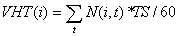[Equation 34]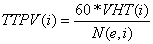[Equation 35]

Where:

• VHT = Total vehicle-hours traveled on link "i" (veh-min).
• TTPV = Total time per vehicle (sec/veh).
• TS = Duration of time slice, 1.0 seconds in CORSIM.
• N(i,t) = Number of vehicles present on link "i" at time slice "t."
• N(e,i) = Number of vehicle exiting the link during simulation period.

The Total Time per Vehicle is computed by dividing the total time by the number of vehicles exiting the link during the simulation period. Note that vehicles that fail to exit the link during the simulation period will generate total travel time but will not be counted in the number of vehicles exiting the link, thus tending to increase the computed total time per vehicle.

The Average Speed is the ratio of VMT over VHT. VMT is computed by multiplying the number of vehicles exiting the link during the simulation period by the length of the link. Note that it excludes the delay to vehicles denied entry to the network.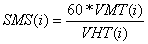[Equation 36]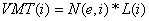[Equation 37]

Where:

• SMS = Mean speed of vehicles on link "i" (mph).
• VHT = Total vehicle-hours traveled on link "i" (veh-min).
• VMT = Total vehicle-miles traveled on link "i" (veh-mi).
• L = Length of link "i" (mi).
• N(e,i) = Number of vehicles exiting link "i."

Note that this equation has the effect of shifting to the next simulation period all of the VMT generated by any vehicles that fail to leave the link during the current simulation period.

The Total Time Rate (min/mi) is the inverse of the mean speed.

##### Computation of Delay

CORSIM divides Total Travel Time into two additive components: Move Time and Delay Time. Move Time is the theoretical time it would take to traverse a link at the free-flow speed (even if the vehicle driver does not really want to go at that speed, some may want to go slower, some may want to go faster). The Total Travel Time is the amount of time it actually takes to traverse a link. The Delay Time is then the difference between the observed Total Travel Time and the theoretical Move Time at the free-flow speed. CORSIM also outputs the Delay Time Rate in terms of minutes of delay per mile traveled.

Move Time is the travel time if all travel was done at the link free-flow speed. It is computed in CORSIM by multiplying the Total Time measured by the ratio of the free-flow speed to the average speed for the link.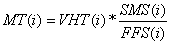[Equation 38]

Where:

• MT = Move Time at free-flow speed on link "i" (veh-min).
• VHT = Total vehicle-hours traveled on link "i" (veh-min).
• SMS = Mean speed of vehicles on link "i" (mph).
• FFS = Free-flow speed on link "i" (mph).

Delay Time is the difference between the total travel time and the Move Time. It does NOT include delay time for vehicles denied entry to the network.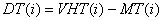[Equation 39]

Where:

• DT = Delay time on link "i" (veh-min).
• VHT = Total vehicle-hours traveled on link "i" (veh-min).
• MT = Move Time at free-flow speed on link "i" (veh-min).

The Delay Time per Vehicle is the Delay Time divided by the number of vehicles exiting the link during the simulation period.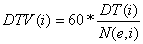[Equation 40]

Where:

• DTV = Delay time per vehicle on link "i" (secs).
• DT = Delay time on link "i" (veh-min).
• N(e,i) = Number of vehicles exiting link "i" during simulation period.

Note that vehicles denied entry to the link are not included in the delay. Note also that vehicles failing to exit the link during the simulation period generate delay that is included in the numerator but the number of these vehicles is not included in the denominator.

The Delay Time Rate (min/mi) is the delay time divided by the VMT.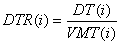[Equation 41]

Where:

• DTR = Delay time rate on link "i" (min/mi).
• DT = Delay time on link "i" (veh-min).
• VMT = Total vehicle-miles traveled on link "i" (veh-mi).

Note that the Delay Time Rate includes all vehicles on the link that generate delay during the current time period in the numerator but may or may not exclude any vehicles failing to exit the link by the end of the current time period from the denominator. [The Users Guide does not explicitly state how measures of effectiveness for vehicles failing to exit the link during the simulation period are accumulated, if at all.] Vehicles denied entry to the network or the link are NOT included in the delay.

For non-freeway links CORSIM also identifies two overlapping (and therefore not additive) components of Delay Time. They are the Queue Time and the Stop Time. A vehicle is in a queue all of the time if it is moving below 3 feet per second (with an acceleration rate of less than 2 fpss), and half of the time if it is moving between 3 and 9 feet per second. The vehicle is "stopped" if its speed is less than 3 feet per second.

The Queue Time and the Stop Time are not additive since they overlap. Stop time is a subset of Queue Time.

The mean queue time and the mean stop time are computed by dividing the total queue time or stop time by the sum of the total number of vehicles exiting the link plus the number of vehicles remaining on the link at the end of the simulation period. [Source for this statement is footnote to CUMULATIVE NETSIM STATISTICS table in CORSIM output.]

Version 5 of TSIS/CORSIM now computes "control delay" for signalized links. Control delay is defined in CORSIM as the difference between the actual travel time through the intersection and the theoretical travel time if the vehicle had been able to continue at its original "operating" speed. Operating speed is defined as the speed of the vehicle prior to the start of deceleration for the signal. Operating speed is lower than the free-flow speed for the link, since it takes into account mid-block congestion effects, unrelated to any signals. The influence area of the signal is defined to start when the vehicle begins to decelerate on a link with a downstream signal and end when the vehicle ceases to accelerate on a link with an upstream signal. [There is some dispute among CORSIM experts as to whether this is indeed an accurate description of how CORSIM actually computes control delay. This description is taken from the users guide, but has not been independently verified by this author.]

Note that Control Delay will therefore exclude delays that occur on links upstream of the link with the traffic signal.

##### Computation of Queue

For all other links CORSIM defines a vehicle as being in a queue when it is moving at less than 3 fps and is accelerating/decelerating by less than 2 fpss. If a vehicle is moving at a speed of between 3 fps and 9 fps it is considered to be in a queue 50 percent of the time (every other second). The "maximum queue" is the largest number of vehicles observed to be queued in a lane during the simulation period. The "mean queue" is the mean number of queued vehicles over the simulation time period with "zero" queues included in the average.

The percent of link storage use is the mean number of vehicles in queue times the mean distance between vehicles divided by the link length times the number of lanes.[Equation 42]

Equation 42. PercentStorage open parenthesis, i, close parenthesis equals the quotient of the product of AveQ open parenthesis, i, close parenthesis times 6 times AVL divided by the product of NL open parenthesis, i, close parenthesis times L open parenthesis, i, close parenthesis.

Where:

• %Storage = Percent of available link storage used.
• AveQ = Mean queue length on link "i."
• AVL = Average vehicle length (ft).
• NL = Number of Lanes, including turn bays.
• L = Length of link.

Phase Failure is the number of cycles during the simulation period that the queue fails to clear a traffic signal. Phase Failure is NOT computed for freeway or ramp links.

Note that the reported queue cannot exceed the length of the link. The percent storage CANNOT exceed 100 percent.

##### Computation of Stops

CORSIM does NOT compute stops for freeway and ramp links. For all other links a vehicle is recorded as "stopped" if its speed is less than three feet per second.

##### Computation of Density

CORSIM does not provide density for non-freeway links.

The average content of the link is the cumulative sum of the vehicle-seconds travel time accumulated on the link divided by the cumulative number of seconds in the simulation period. [It is not clear whether the accumulated VHT at the end of the period is used rather than a running total of vehicle-seconds on the link to compute density. We have chosen to show the formula assuming that CORSIM uses the sum of the running total of vehicle-seconds.][Equation 43]

Equation 43. Density open parenthesis, i,T close parenthesis equals the quotient of the product of the quotient of 1 divided by T, times the summation over t of the product of N open parenthesis, t, close parenthesis times TS, divided by the product of L open parenthesis, i, close parenthesis, times NL open parenthesis, i, close parenthesis.

Where:

• Density = Mean density of link "i" over time period "T" (veh/lane-mi).
• T = Length of time period (secs).
• N = Number of vehicles on link at time "t."
• TS = Length of time step (= 1.0 secs in CORSIM).
• NL = Number of Lanes, including turn bays.
• L = Length of link.
##### Computation of Travel-Time Variance

CORSIM does not compute the variance in travel time. The model can be run several times with different random number seeds to obtain a variance. A TSIS output processor can be used to make the computations from the runs. However, the computed variance would not include the impacts of variation in demand or capacity reducing incidents on the facility.

#### 4.1.6 Vissim

This section describes how Vissim computes its measures of effectiveness based upon its Users Guide [Vissim 4.1 User Manual, PTV, Planung Transport Verkehr AG, Karlsruhe, Germany, March 2005.] and additional information from the vendor.

MOEs can be collected by Vissim at detector locations or for selected series of links. MOEs also can be gathered for paths.

• Density (veh/km);
• Speed;
• Volume; and
• Lost Time (Delay).

Node Analysis can produce:

• Volume;
• Average Delay;
• Standing Time;
• Number of Stops; and
• Queue Length.

The Network Performance Evaluation will produce the following systemwide MOEs:

• Number of Vehicles Left in Network;
• Number of Vehicles Exiting Network;
• Total Path Distance (VMT);
• Total Travel Time (VHT);
• Average Speed (mph);
• Total Delay (hrs);
• Stopped Delay (hrs);
• Average Stopped Delay;
• Number of Stops; and
• Average Number of Stops.
##### Computation of HCM Level of Service

Vissim does not directly output HCM Level of Service.

##### Computation of Volume Capacity Ratio

Vissim does not directly output volume/capacity ratios.

##### Computation of Speed and Travel Time

The computation of speed (in the link segment evaluation) follows the general space-time definition of average speed:[Equation 44]

Where:

•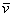= Average speed in the link segment during the analysis period.
• xx(i) = Total distance traveled by vehicle i in the link segment during the analysis period.
• tt(i) = Total time spent by vehicle i in the link segment during the analysis period.

Average speed in the network performance evaluation is defined the same way (total miles traveled divided by total hours spent).

Vissim outputs travel-time results between selected points on the network. The average is computed from the actual travel times of all vehicles that have passed the destination point after they have passed the start point. Vehicles that have not reached the destination point and denied entry vehicles are not included in the travel-time results.

##### Computation of Delay

Vissim defines delay as the difference between the actual vehicle travel time and its desired travel time. Note that this is a microscopic definition of delay that is specific to each vehicle. This is different than the other software packages that compare actual travel times to an average link free-flow travel time.

The average total delay per vehicle is computed only for the vehicles completing the travel-time measurement section. The delay is computed for each vehicle by subtracting the ideal travel time from the actual travel time. The ideal travel time is computed assuming no other vehicles on the network and no delays at signal controls or stop signs. Reduced speeds for turns are taken into account in the ideal travel time.

##### Computation of Queue

Vissim allows the user to define a queue according to the maximum vehicle speed for the beginning of the queue (default is 5 km/hr), the minimum vehicle speed at its end (default is 10 km/hr), and the maximum spacing between vehicles (default is 20 meters).

Vissim allows the user to identify a queue counter location. The distance to the farthest upstream point of any queue at this location is tallied. If the queue backs up onto multiple links, the longest distance is recorded. If the front of the queue begins to discharge Vissim keeps tracking to the back of the queue until no queued vehicles remain between the queue counter location and the current back of queue.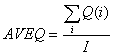[Equation 45]

Where:

• AVEQ = Average Back Of Queue over analysis period (meters).
• Q(i) = Observed Back of Queue length (meters) at end of time step (i).
• I = Total number of time steps in analysis period.

Incidentally, if the queue extends from one queue counter location back to another, the queue length is truncated to that distance.

Vissim also will report the number of stops within the queue (which are defined as situations when the vehicle enters the queue, i.e., when its speed falls below the speed for the beginning of the queue).

##### Computation of Stops

Stops in the delay and node evaluation are defined as situations when the vehicle's speed falls to zero.

##### Computation of Density

The computation of density (in the link segment evaluation) follows the general space-time definition of traffic density: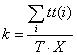[Equation 46]

Where:

• k = Average density in the link segment during the analysis period.
• tt(i) = Total time spent by vehicle i in the link segment during the analysis period.
• T = Duration of the analysis period.
• X = Length of the link segment.
##### Computation of Travel-Time Variance

Vissim does not directly output travel-time variance information, however; the software provides a raw data file with all single travel times of the individual vehicles. These can be used to determine the variance.

#### 4.1.7 Paramics

This section discusses the MOEs produced by Quadstone Paramics Version 5.0 (2004). [ Quadstone Paramics V5.0 Modeler Reference Manual, Quadstone Limited, Edinburgh, Scotland, August 2004.]

Paramics produces systemwide and link-specific MOEs.

Systemwide MOEs (Economic Info) that are reported for every minute of the simulation include:

• Number of Vehicles currently on network;
• Mean speed of vehicles currently on network at specific point in time;
• Cumulative number of vehicles simulated on network;
• Accumulated Total Travel Time (VHT) since start of simulation;
• Accumulated Total Distance Traveled (VMT) since start of simulation;
• Mean Speed of all vehicles on network since start of simulation;
• Total number of stops made by public transit vehicles;
• Total time stopped for public transit;
• Passengers Boarding; and
• Passengers Alighting.

Paramics also outputs performance measures by trip (from origin to destination) and for public transit vehicles.

• Turn counts/flows/proportions;
• Percentage time delay;
• Queues Max/Average;
• Blocking;
• OD Data;
• Journey times;
• User-defined performance criteria; and
• LOS, MOEs, intersection LOS.
##### Computation of HCM Level of Service

The Paramics Analyzer does output direct level of service grades for individual links. These LOS grades are based upon the threshold numbers given in the HCM. [ Quadstone Paramics V5.0, Analyser Reference Manual, Quadstone Limited, Version No. 1.0, July 21, 2004.]

Vehicle densities are converted to passenger car units using PCE s provided by the analyst. The PCU densities are then used to look up the HCM LOS grade using analyst editable look-up tables.

The Paramics Analyzer can compute Percent Time Delay for use in estimating LOS for two-lane rural roads. However, since Paramics does not simulate passing maneuvers using opposing lanes, it would be most reliable for 2-lane roads with 100 percent no-passing zones.

The Node LOS is the flow weighted average of the link approach LOSs. The flow weights are the number of vehicles exiting each link approach to the node during the analysis period. Note that PCU s are vehicles multiplied by their appropriate PCE. The PCE s are fixed equivalencies input by the analyst by vehicle-type.

##### Computation of Volume Capacity Ratio

Paramics does not directly output volume/capacity ratios.

##### Computation of Speed and Travel Time

Paramics outputs system-level VMT, VHT, and mean speed accumulated by one-minute periods throughout the simulation period. These results exclude delays incurred by vehicles denied entry to the network during any part of the simulation period.

Link speed is equal to the link length divided by the actual travel time = VMT/VHT. The delays incurred by vehicles denied entry to the link during any part of the simulation period are excluded from this computation.

Link VMT and VHT are not directly output by Paramics. The link level outputs of flow and speed can be combined with the link length inputs to obtain VMT and VHT by link.

Mean link travel times are not directly output by Paramics but can be computed from the link level outputs of flow, speed, and link lengths.

##### Computation of Delay

Paramics computes delay as the difference between the cumulative travel time of all vehicles present on a link at some point within each one-minute time period, and the theoretical travel time for those vehicles if they had traveled at the user coded free-flow speed for the link. Paramics does not break down the delay into different components.

Link delay is the difference between the actual travel time and the ideal travel time.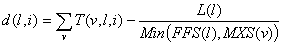[Equation 47]

Equation 47. d open parenthesis, l,i, close parenthesis equals the difference of the summation over v of T open parenthesis, v,l,i, close parenthesis minus the quotient of L open parenthesis, l, close parenthesis divided by the minimum of either FFS open parenthesis, l, close parenthesis, or MXS open parenthesis, v, close parenthesis.

Where:

• d(l) = Total Delay for link (l) (veh-hrs) during interval (i).
• T(v,l,i) = Actual travel time for vehicle (v) exiting link (l) during interval (i)(hrs).
• FFS(l) = Free-flow speed for link (l) (mph).
• MXS(v) = Maximum speed for vehicle (v) (mph).
• L(l) = Length of link (l) (miles).

Average link stop time is the mean time spent stopped for vehicles exiting the link during the analysis period.

##### Computation of Queue

Paramics allows a user to select their own definition of a queue according to the maximum vehicle speed and a maximum distance between vehicles. The defaults are 2 meters per second (for speed), and 10 meters between vehicles. The minimum number of vehicles before Paramics records them as a queue also can be set by the analyst (page 14 Analyzer Manual). The queue is reported on a second by second basis in terms of number of vehicles and in terms of the starting distance and ending distance within the link.

Paramics Analyzer reports the mean and maximum queue observed over the simulation time period (or a user specified time period). The queue must meet the minimum number of vehicles thresholds selected by the analyst to be identified as a queue and reported.[Equation 48]

Where:

• AVEQ = Average Queue over analysis period.
• Q(i) = Observed Queue at end of time step (i).
• I = Total number of time steps in analysis period.

At the end of each time step the vehicles in queue are converted to a queue length by multiplying each by its appropriate PCE and summing the lengths of the vehicles. The spacing in between vehicles is NOT included in the queue length (see page 21, Analyzer Users Manual).

Note that this mean queue will be different than that for signals, since this mean queue includes time steps when the signal is green as well as red, while the signal mean queue is limited to time steps when the signal is red.

Note that the maximum queue cannot be longer than the length of the link. The Average Queue will be impacted by this limitation on the maximum queue. [The SIAS version of Paramics does have a queue tracker that will track a queue back over several links.]

##### Computation of Stops

Paramics does not report the number of stops on the link by non-public transit vehicles.

##### Computation of Density

Paramics computes the mean density in terms of passenger car units per lane-mile over an analysis period as the sum of the average density during the simulation period and the density of vehicles remaining at the end of the period.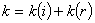[Equation 49]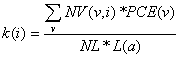[Equation 50]

Equation 50. k open parenthesis, i, close parenthesis equals the quotient of the summation over v of NV open parenthesis, v,i, close parenthesis times PCE open parenthesis, v, close parenthesis, divided by the product of NL times L open parenthesis, a, close parenthesis.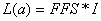[Equation 51]

Where:

• k = Reported link density for analysis period (pcu/lane-mile).
• k(i) = Computed link density over analysis period (i).
• k(r) = Computed density of vehicles remaining on link at end of analysis period.
• NV(v,i) = Cumulative number of vehicles of type (v) exiting the link during period (i).
• PCE(v) = Passenger car equivalence of vehicle type (v).
• NL = Number of lanes on link.
• L(a) = Available distance on link (mile-hours).
• FFS = Free-flow speed on link (mph).
• I = Duration of analysis period (hrs).

The density for the remaining vehicles is computed using the same formula as for computing the link density during the analysis period, substituting the PCU s remaining for the PCU s accumulated during the analysis period in the formula.

The analyst can exclude the "remainder" vehicles from the density calculation, if desired.

##### Computation of Travel-Time Variance

Paramics does not directly output travel-time variance information, however; the software can be executed multiple times to determine the variance of the mean travel time for each run. An analyzer program provided with Paramics can be used to compute the variance.

#### 4.1.8 Aimsun

This discussion is based upon an excerpt of the Aimsun Users Guide currently under preparation that was advanced to Dowling Associates on January 13, 2006 by the developers of Aimsun (Dr. Jaume Barcelo and Pablo Barcelo). The equations shown below are quoted from the draft Aimsun Users Guide.

Aimsun outputs flow, speed, density, travel time and delay measures of effectiveness for several different levels of aggregation: whole system, individual links (called sections in Aimsun), series of links, and turning movement. The MOEs can be output by trip origin and destination (OD). Public transport performance outputs also are provided.

Regardless of level of aggregation the following outputs can be generated:

• Mean Flow;
• Density;
• Mean Speed;
• Harmonic Mean Speed;
• Total Travel Distance (VMT);
• Total Travel Time (VHT);
• Mean Travel Time;
• Mean Delay Time;
• Stop Time;
• Number of Stops;
• Fuel Consumed; and
• Pollutants Emitted.

For individual links or series of links the above MOEs are provided plus the following additional outputs:

• Mean Queue Length (veh/lane); and
• Maximum Queue Length (veh/lane).

For OD trip information the general MOEs (listed above for all levels of aggregation) are produced plus the following additional outputs:

• Lost Vehicles – "Total number of vehicles that have been lost while trying to do the trip from origin to destination, and which have therefore not reached the correct destination."

The analyst also can customize the calculation of additional MOEs. Detectors also can be placed on the network to gather point MOEs.

##### Computation of HCM Level of Service

Aimsun does not directly output HCM level of service.

##### Computation of Volume Capacity Ratio

Aimsun does not directly output volume/capacity ratios.

##### Computation of Speed and Travel Time

For system-level MOEs Aimsun accumulates the following statistics for each vehicle in the network.

The total system vehicle travel time (VHT) is computed as follows. Note that only vehicles able to exit the system during the simulation period generate system travel time. Vehicles remaining in the system are excluded from the total system travel-time computation.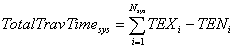[Equation 52]

Where:

• TENi = Entrance time of vehicle (i) in the system (seconds).
• TEXi = Exit time of vehicle (i) from the system (seconds).
• Nsys = Number of vehicles that exit the system during period I.

The total travel distance (VMT) is computed as follows. Note that only vehicles able to exit the system during the simulation period generate system travel distance. Vehicles remaining in the system are excluded from the total system travel distance computation.[Equation 53]

Where:

• Di = Total Distance traveled by vehicle (i) in the system (meters). [Although only metric are shown here, Aimsun accumulates all measures in the native unit system in which the network is coded, U.S. Customary or Metric units.]
• Nsys = Number of vehicles that exit the system during period I.

Two averages are computed for mean system speed. One is the arithmetic average. The other is the harmonic mean.[Equation 54][Equation 55]

Where:

• Ssys = Arithmetic Average Speed per vehicle (Km/h).
• HSsyss= Harmonic mean Speed per vehicle (Km/h).
• Nsys = Number of vehicles that exit the system during period I.
• Si = Spot speed of vehicle (i) (Km/h).

For individual links the total distance and time traveled are computed as follows. Note that vehicles failing to exit the link during the simulation period are not counted.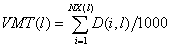[Equation 56]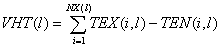[Equation 57]

Where:

• VMT(l) = Total distance traveled by all the vehicles (i) exiting the link (l) (Km).
• VHT(l) = Total travel time experienced by all exiting vehicles (i) on link (l) (seconds).
• Di = Distance traveled by exiting vehicle (i) while on link (l).
• NX(l) = Number of vehicles exiting link (l) during simulation period.

The arithmetic average vehicle speed for the link is computed as follows.[Equation 58]

Equation 58. S open parenthesis, l, close parenthesis equals the product of 3.6 times the quotient of the summation from i equals 1 to NX open parenthesis, l, close parenthesis of the quotient of the sum of LS open parenthesis, l, close parenthesis plus LT open parenthesis, l, close parenthesis, divided by the difference of TEX open parenthesis, i,l, close parenthesis minus TEN open parenthesis, i,l, close parenthesis.
• S(l) = Arithmetic Average Speed per exiting vehicle of a link (Km/h).
• TEN(i,l) = Entrance time of vehicle (i) to link (l) (seconds).
• TEX(i,l) = Exit time of vehicle (i) from link (l) (seconds).
• LS(l) = Length of link (m).
• LT(l) = Length of turn (m).
• NX(l) = Number of vehicles exiting link (l) during simulation period.

A harmonic mean vehicle speed for vehicles exiting the link also is computed.

##### Computation of Delay

For individual links the delay is computed as follows.[Equation 59]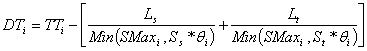[Equation 60]

Equation 60. DT subscript i equals the difference of TT subscript i minus the sum of the quotient of L subscript s divided by the minimum of either SMax subscript i or the product of S subscript s times theta subscript i, plus the quotient of L subscript t divided by the minimum of either SMax subscript I or the product of S subscript t times theta subscript i.

Where

• DTsec = Average Delay Time per vehicle of a section.
• DTi = Average section delay time of vehicle (i) (seconds).
• TTi = The difference between the link exit time and the link entrance time for vehicle (i).
• Ss = Speed limit of link (m/s).
• St = Speed limit of turning (m/s).
• Θi = Ratio of Speed Limit accepted by vehicle i.
• SMaxi = Maximum desired Speed of vehicle i (m/s).
• Ls = Distance of link (meters).
• Lt = Distance of turning (meters).
• NX(l) = Number of vehicles exiting link (l) during simulation period.

Stop time also is computed. The Users Guide does not identify whether or not slow vehicles are counted as stopped or not.

##### Computation of Queue

For individual links the average and maximum queue lengths in terms of vehicles are computed as follows. A similar formula is used for measuring queues for individual turning movements.[Equation 61]

Equation 61. AQL subscript sec equals the quotient of the summation over l belongs to sec of the quotient of the summation over t subscript i belongs to T subscript l of the product of QL subscript l,t subscript open parenthesis, i minus 1, close parenthesis, times the difference of t subscript l,i, minus t subscript l, open parenthesis, i minus 1, close parenthesis, divided by I, divided by NBLanes subscript sec.[Equation 62]

• AQL = Average Queue Length (vehicles).
• QLl,t = Queue Length in the lane l at time t (veh).
• MaxQLl = Maximum Queue Length in the lane l (veh).
• I = Interval of statistics (seconds).
• Tl = (0, t l,1,..., t l,m, I): Instants when the queue length in lane l changes.
• NBLanessec = Number of lanes of link (section).

A vehicle is considered "queued" and therefore "stopped" when its speed below the user-specific queue threshold speed. A vehicle is not considered as having left the queue until its speed exceeds the user specified "queue leaving speed."

##### Computation of Stops

For individual links the average number of stops is computed as follows.[Equation 63]

Equation 63. NS open parenthesis, l, close parenthesis equals the quotient of the summation from i equals 1 to NX open parenthesis, l, close parenthesis of TNS open parenthesis, l, close parenthesis, divided by NX open parenthesis, l, close parenthesis.
• NS(l) = Average Number of Stops per exiting vehicle on link (l).
• TNS(l) = Total Number of stops accumulated on link by exiting vehicle (i).
• NX(l) = Number of vehicles exiting link (l) during simulation period.

A vehicle is considered "stopped" when its speed falls below the user-specific queue threshold speed. A vehicle is not considered as having left the queue, (and therefore cannot generate additional stops) until its speed exceeds the user specified "queue leaving speed."

##### Computation of Density

The following equation is used to compute mean density of vehicles on a link.[Equation 64]

Equation 64. k open parenthesis, sect, close parenthesis equals the product of 1000 times the quotient of the summation over l belongs to sec of the quotient of the summation over t subscript i belongs to T subscript l of the product of NVeh subscript l,t subscript open parenthesis, i minus 1, close parenthesis, times the difference of t subscript l,i, minus t subscript l, open parenthesis i minus 1, close parenthesis, divided by I, divided by the summation over l belongs to sec of L subscript l.

Where:

• K(sect) = Density for section (link) (veh/lane-m).
• Ll = Length of lane l (m).
• NVehl,t = Number of vehicles in the lane l at time t.
• I = Interval of statistics (seconds).
• Tl = (0, tl, ,..., tl,m, I): Instants when the number of vehicles in lane l changes.
##### Computation of Travel Time Variance

Aimsun does not directly output travel time variance information, however; the software can be executed multiple times to determine the variance of the mean travel time for each run.

#### 4.1.9 S-Paramics

This section discusses the MOEs produced by S-Paramics Version 2005.1. This section has been provided to the author by the vendor. The author respects the expertise of the people who have provided this description, and the section has been incorporated for that reason. However, the author has not independently verified the accuracy of this description.

Paramics produces systemwide, link-specific, path defined, OD and point-specific MOEs.

Systemwide MOEs (Economic Info) that are reported for every minute of the simulation include:

• Number of Vehicles currently on the network;
• Mean Speed of vehicles currently on network at specific point in time;
• Mean Time Traveled reported as mean delay;
• Accumulated Total Distance Traveled (VMT) since start of simulation;
• Total number of vehicles passed through the network;
• Mean Speed of all vehicles on network since start of simulation; and
• Bus statistics, including bus stopped time and number of passengers on or off.

For individual links the following MOE outputs are provided:

• Number of vehicles traveling the link in the period;
• Flow count normalized to vehicles per hour;
• Accumulated time on the link in the period;
• Number of vehicles at present on the link;
• Mean speed on the link;
• Turn counts and flows;
• Density;
• Emissions; and
• Queue transitions and queued time.

For user-defined paths the following queue MOE outputs are available:

• Minimum, average and maximum vehicles and PCUs queued along the path in a user-defined interval;
• Minimum, average and maximum length of the queue in meters;
• Path length;
• Minimum, average and maximum journey time; and

The following point data is available, for which histogram distributions are produced:

• Flow;
• Speed;
• Acceleration.

Lane use and lane changes also may be measured on a per link basis.

##### Computation of HCM Level of Service

S-Paramics does not directly output level of service.

##### Computation of Volume Capacity Ratio

S-Paramics does not directly output volume/capacity ratios.

##### Computation of Speed and Travel Time

Paramics outputs network level total distance VMH, mean travel time (defined as "mean delay" in S-Paramics) and mean speed, all accumulated by one-minute periods throughout the simulation period. Total travel time, VHT, is calculated by multiplying the mean travel time by the total number of completed trips.

These results exclude vehicles denied entry to the network during any part of the simulation period. However, these vehicles are accounted for separately as off network queue time, collected at one-minute periods throughout the simulation period.

Link VMT and VHT are not directly output by Paramics. The link level outputs of flow and speed can be combined with the link length to obtain VMT and VHT by link. The delays incurred by vehicles denied entry to the link during any part of the simulation period are excluded from this computation.

##### Computation of Delay

S-Paramics does not directly output delay.

##### Computation of Queue

Paramics allows a user to select their own definition of a queue according to the maximum vehicle speed and a maximum distance between vehicles. The defaults are 2 meters per second (for speed), and 10 meters between vehicles. The minimum number of vehicles before Paramics records them as a queue also can be set by the analyst. Additionally, the analyst selects the appropriate method to handle multiple queues occurring on the same link and lane.

S-Paramics reports the mean and maximum queue observed over the simulation time period. The queue must meet the minimum number of vehicles thresholds selected by the analyst to be identified as a queue.

Once a vehicle has entered a queue by going below a specified head way and/or speed it remains in the queued state until the headway and/or speed rise above another set of values. Hysteresis is built into the system.

The average queue is computed by the following equation. The end of the queue is defined as the position of the tail of the last vehicle in the queue this may be output by lane or over the link. The average queue may be computed for every time spent in the reporting period or just by those when there was a queue. Including the times when no queue was present will bias the result to a lower value.[Equation 65]

Where:

• AVEQ = Average Queue over analysis period.
• Q(i) = Observed Queue at end of time step (i).
• I = Total number of time steps in analysis period.

At the end of each time step the vehicles in queue are converted to a queue length by multiplying each by its appropriate PCE and summing the lengths of the vehicles. Note that this mean queue will be different than that for signals, since this mean queue includes time steps when the signal is green as well as red, while the signal mean queue is limited to time steps when the signal is red.

It also should be noted that the queue is reported based on user-defined paths, which may span many links, hence the maximum and average queue is not limited by the length of links.

S-Paramics reports the number of times a vehicle enters a queued state on each link and the time spent in this state.

#### Computation of Stops

S-Paramics reports the number of transitions into a queued state and the time spent in that state per link.

#### Computation of Density

Paramics computes the mean density in terms of passenger car units per lane-mile over an analysis period as the sum of the average density during the simulation period and the density of vehicles remaining at the end of the period.[Equation 66][Equation 67]

Equation 67. K open parenthesis, i, close parenthesis equals the quotient of the summation over v of the product of NV open parenthesis v,i, close parenthesis times PCE open parenthesis, v, close parenthesis, divided by the product of NL times L open parenthesis, a, close parenthesis.[Equation 68]

Where:

• k = Reported link density for analysis period (pcu/lane-mile).
• k(i) = Computed link density over analysis period (i).
• k(r) = Computed density of vehicles remaining on link at end of analysis period.
• NV(v,i) = Cumulative number of vehicles of type (v) exiting the link during period (i).
• PCE(v) = Passenger car equivalence of vehicle type (v).
• NL = Number of lanes on link.
• L(a) = Available distance on link (mile-hours).
• FFS = Free-flow speed on link (mph).
• I = Duration of analysis period (hrs).

The density for the remaining vehicles is computed using the same formula as for computing the link density during the analysis period, substituting the PCU s remaining for the PCU s accumulated during the analysis period in the formula.

The analyst can exclude the "remainder" vehicles from the density calculation if desired.

##### Computation of Travel Time Variance

S-Paramics reports travel times between OD pairs and also travel times along specified paths in the model. As each individual vehicle is logged, variance can be reported within each run and across a number of runs.

### 4.2 Test Bed Results

The following tools were tested on a test network to observe how the computations of MOEs varies between the tools: HCS, Synchro, SimTraffic, CORSIM, Vissim, Q-Paramics, S-Paramics, and Aimsun.

The Vissim results produced by Dowling Associates were proofed and corrected by PTV personnel. [Initial results indicated a problem with the Synchro to Vissim conversion software provided by Vissim. There was also a problem with how Dowling had selected the node data collection box in Vissim. PTV made the necessary corrections and returned both input and output files to Dowling Associates.]

Q-Paramics is the Quadstone version of Paramics. S-Paramics is the SIAS version of Paramics. The Q-Paramics results were produced by Dowling Associates. The S-Paramics results were provided by SIAS consultancy. Dowling Associates has not proofed or verified these results, but respects the expertise of the people that produced these results, and has included these results on that basis.

The Aimsun results were provided by Dr. Jaume Barcelo, since Dowling Associates lacks licenses and experienced personnel for operating Aimsun. Dowling Associates has not proofed or verified these results, but respects the expertise of the people that produced these results, and has included these results on that basis.

Dynasmart-P was not tested because Dowling Associates lacks licenses and experienced personnel on this tool.

##### Test Network

The test network consists of a freeway and an arterial street. They are not connected. They operate independently.

The freeway is a single (right-bound) direction of freeway, 2.75 miles long, 3-lanes wide, with a single-lane on-ramp and a single-lane off-ramp (see Figure 1).

Figure 1. Test Freeway FacilityThe arterial street is bidirectional, one-mile-long, with a single lane in each direction, and two signalized intersections (see Figure 2).

Figure 2. Test Arterial StreetAt each intersection the approach widens to a single exclusive left turn lane, a single exclusive through lane, and a single exclusive right turn. Both signals are 100-second cycle fixed time with exclusive left turn phases. Each left and through signal phase gets the same amount of green time (see Figure 3). Right turns on red are prohibited.

Figure 3. Signal Timing for Example Signal on Test Arterial

Timing Window

East-Bound Left

East-Bound Through

East-Bound Right

West-Bound Left

West-Bound Through

West-Bound Right

North-Bound Left

North-Bound Through

North-Bound Right

South-Bound Left

South-Bound Through

South-Bound Right

Lanes and Sharing (#RL)

Left

Through

Right

Left

Through

Right

Left

Through

Right

Left

Through

Right

Traffic Volume (vph)

100

100

100

100

100

100

100

100

100

100

100

100

Turn Type

Prot

No value

Prot

Prot

No value

Prot

Prot

No value

Prot

Prot

No value

Prot

Protected Phases

7

4

4

3

8

8

5

2

2

1

6

6

Permitted Phases

No value

No value

No value

No value

No value

No value

No value

No value

No value

No value

No value

No value

Detector Phases

7

4

4

3

8

8

5

2

2

1

6

6

Minimum Initial(s)

4.0

4.0

4.0

4.0

4.0

4.0

4.0

4.0

4.0

4.0

4.0

4.0

Minimum Split(s)

8.0

21.0

21.0

8.0

21.0

21.0

8.0

21.0

21.0

8.0

21.0

21.0

Total Split(s)

24.0

26.0

26.0

24.0

26.0

26.0

24.0

26.0

26.0

24.0

26.0

26.0

Yellow Time(s)

3.0

3.0

3.0

3.0

3.0

3.0

3.0

3.0

3.0

3.0

3.0

3.0

All-Red Time(s)

0.0

2.0

2.0

0.0

2.0

2.0

0.0

2.0

2.0

0.0

2.0

2.0

Lag

Lag

Lag

Lag

Lag

Lag

Lag

Lag

Yes

Yes

Yes

Yes

Yes

Yes

Yes

Yes

Yes

Yes

Yes

Yes

Recall Mode

Max

Max

Max

Max

Max

Max

Max

Max

Max

Max

Max

Max

Actuated Effect. Green(s)

21.0

21.0

21.0

21.0

21.0

21.0

21.0

21.0

21.0

21.0

21.0

21.0

Actuated g/C Ratio

0.21

0.21

0.21

0.21

0.21

0.21

0.21

0.21

0.21

0.21

0.21

0.21

Source: Screenshot of Synchro Input Screen.

For the purpose of comparing HCM results to simulation results, the peak-hour factors within HCS and Synchro have been set at 1.00 for all movements. The percent heavy vehicles was set at 0 percent.

While some of these assumptions would be unusually simplistic for U.S. practice, they have been selected to dampen as much as possible the differences in how the various simulation models reflect vehicle behavior and signal operation. The attempt with this simple test network is to obtain very simplistic vehicle behavior so that observed differences in the MOEs produced by the tools are mostly the result of differences in how the MOEs are computed and to a lesser extent the result of how vehicle behavior and signal operation are modeled within each tool.

##### Comparison of MOEs Produced Under Free-Flow Conditions

The MOEs computed by the various tools were first tested under free-flow conditions. The freeway mainline volume was 900 vph entering the study section. Each freeway on-ramp and off-ramp carried a volume of 100 vph. Each turning movement of each leg of each signalized intersection on the test arterial was assumed to serve a demand of 100 vph. Thus each intersection carries a total 1,200 vph, summed over all 4 approaches.

Table 15. Basic Freeway Section Results – Free-Flow

Freeway Section

HCS

Synchro

SimTraffic

CORSIM

Vissim

Q-Paramics

Aimsun

S-Paramics

Vehicles Enter

900

Not applicable
[Not directly output by the tool. Computed manually by the analyst based on other outputs provided by the tool.]

893

Data not readily available in the standard output for a specific link.
[Not directly output by the tool. Computed manually by the analyst based on other outputs provided by the tool.]

Data not readily available in the standard output for a specific link.
[Not directly output by the tool. Computed manually by the analyst based on other outputs provided by the tool.]

911

904

901

Vehicles Exit

900
[Not directly output by the tool. Computed manually by the analyst based on other outputs provided by the tool.]

Not applicable
[Not directly output by the tool. Computed manually by the analyst based on other outputs provided by the tool.]

896

907

886

921

886

900

SMS (mph)

60.0

Not applicable
[Not directly output by the tool. Computed manually by the analyst based on other outputs provided by the tool.]

52.0

59.4

59.1

62.4

62.4

65.3

Density (v/ln-mi)

5.0

Not applicable
[Not directly output by the tool. Computed manually by the analyst based on other outputs provided by the tool.]

5.7
[Not directly output by the tool. Computed manually by the analyst based on other outputs provided by the tool.]

5.1

5.0

5.0

4.7

4.6

HCM LOS

A

Not Applicable
[Not directly output by the tool. Computed manually by the analyst based on other outputs provided by the tool.]

A
[Not directly output by the tool. Computed manually by the analyst based on other outputs provided by the tool.]

A
[Not directly output by the tool. Computed manually by the analyst based on other outputs provided by the tool.]

A
[Not directly output by the tool. Computed manually by the analyst based on other outputs provided by the tool.]

A
[Not directly output by the tool. Computed manually by the analyst based on other outputs provided by the tool.]

A
[Not directly output by the tool. Computed manually by the analyst based on other outputs provided by the tool.]

A
[Not directly output by the tool. Computed manually by the analyst based on other outputs provided by the tool.]

• All models were coded with a 60 mph free-flow speed and a demand of 900 vehicles per hour. According to HCM, the 3-lane freeway segment is at 14 percent of capacity at this level of demand.
• All simulation tool results are the average of five runs with varying random number seeds.
• Simulation tools not calibrated. All parameters at default. Note that Aimsun, Q-Paramics, and S-Paramics were produced by their respective vendors and not the authors of this report. Note that Vissim results are based on corrections to author's initial coding by the vendor.
• Synchro does not output freeway performance results.

Table 16. Freeway Facility Results – Free-Flow

Freeway Section

HCS

Synchro

SimTraffic

CORSIM

Vissim

Q-Paramics

Aimsun

S-Paramics

Vehicles Enter

1,000

Not Applicable
[Not directly output by the tool. Computed manually by the analyst based on other outputs provided by the tool.]

999

Data not readily available in the standard output for a specific link.
[Not directly output by the tool. Computed manually by the analyst based on other outputs provided by the tool.]

Data not readily available in the standard output for a specific link.
[Not directly output by the tool. Computed manually by the analyst based on other outputs provided by the tool.]

1,022

1,007

1,029

Vehicles Exit

1,000
[Not directly output by the tool. Computed manually by the analyst based on other outputs provided by the tool.]

Not Applicable
[Not directly output by the tool. Computed manually by the analyst based on other outputs provided by the tool.]

996

999

987

1,018

956

985

VMT (v-mi)

2,587

Not Applicable
[Not directly output by the tool. Computed manually by the analyst based on other outputs provided by the tool.]

2,583

2,597

2,599

2,805
[Not directly output by the tool. Computed manually by the analyst based on other outputs provided by the tool.]

2,833

2,700

VHT (v-h)

43.3

Not Applicable
[Not directly output by the tool. Computed manually by the analyst based on other outputs provided by the tool.]

49.0

43.8

44.3

45.1
[Not directly output by the tool. Computed manually by the analyst based on other outputs provided by the tool.]

45.7

40.7

SMS (mph)

59.7

Not Applicable
[Not directly output by the tool. Computed manually by the analyst based on other outputs provided by the tool.]

52.7

59.3

58.6

62.2

61.9

64.6

• All models were coded with a 60 mph free-flow speed and a demand of 1,000 vehicles per hour (combination of mainline in and on-ramp volumes).
• All simulation tool results are the average of five runs with varying random number seeds.
• Simulation tools not calibrated. All parameters at default. Note that Aimsun, Q-Paramics, and S-Paramics were produced by their respective vendors and not the authors of this report. Note that Vissim results are based on corrections to author's initial coding by the vendor.
• Synchro does not output freeway performance results.

Table 17. Signalized Intersection Results – Free-Flow

Intersect MOEs

HCS

Synchro

SimTraffic

CORSIM

Vissim

Q-Paramics

Aimsun

S-Paramics

Vehicles Enter

1,200

1,200

1,200

Data not readily available in the standard output for a specific link.
[Not directly output by the tool. Computed manually by the analyst based on other outputs provided by the tool.]

Data not readily available in the standard output for a specific link.
[Not directly output by the tool. Computed manually by the analyst based on other outputs provided by the tool.]

Data not readily available in the standard output for a specific link.
[Not directly output by the tool. Computed manually by the analyst based on other outputs provided by the tool.]

1,222

1,198

Vehicles Exit

1,200
[Not directly output by the tool. Computed manually by the analyst based on other outputs provided by the tool.]

1,200
[Not directly output by the tool. Computed manually by the analyst based on other outputs provided by the tool.]

1,208

1,199

1,225

1,197

1,198

1,201

Delay/Vehicle(s)

34.9

37.5

38.1

31.8

29.0

26.4

30.6

30.1

EBT Q 50% (ft)

63
[Not directly output by the tool. Computed manually by the analyst based on other outputs provided by the tool.]

54

61

25
[Not directly output by the tool. Computed manually by the analyst based on other outputs provided by the tool.]

17

29

22
[Not directly output by the tool. Computed manually by the analyst based on other outputs provided by the tool.]

90

HCM LOS

C

D

D
[Not directly output by the tool. Computed manually by the analyst based on other outputs provided by the tool.]

C
[Not directly output by the tool. Computed manually by the analyst based on other outputs provided by the tool.]

C
[Not directly output by the tool. Computed manually by the analyst based on other outputs provided by the tool.]

C
[Not directly output by the tool. Computed manually by the analyst based on other outputs provided by the tool.]

C
[Not directly output by the tool. Computed manually by the analyst based on other outputs provided by the tool.]

C
[Not directly output by the tool. Computed manually by the analyst based on other outputs provided by the tool.]

• All models were coded with a demand of 1,200 vehicles per hour for the intersection (100 vph for each of the 12 turn movements. According to HCM, the signal is at 28 percent of capacity).
• All simulation tool results are the average of five runs with varying random number seeds.
• Simulation tools not calibrated. All parameters at default. Note that Aimsun, Q-Paramics, and S-Paramics were produced by their respective vendors and not the authors of this report. Note that Vissim results are based on corrections to author's initial coding by the vendor.

Table 18. Arterial Street Results – Free-Flow

EB Arterial

HCS

Synchro

SimTraffic

CORSIM

Vissim

Q-Paramics

Aimsun

S-Paramics

Art Speed mph

23.3

21.7

20.0

21.0

19.0

30.6

26.9

26.0

Art LOS

C

C

C
[Not directly output by the tool. Computed manually by the analyst based on other outputs provided by the tool.]

C
[Not directly output by the tool. Computed manually by the analyst based on other outputs provided by the tool.]

C
[Not directly output by the tool. Computed manually by the analyst based on other outputs provided by the tool.]

A
[Not directly output by the tool. Computed manually by the analyst based on other outputs provided by the tool.]

B
[Not directly output by the tool. Computed manually by the analyst based on other outputs provided by the tool.]

B
[Not directly output by the tool. Computed manually by the analyst based on other outputs provided by the tool.]

• For eastbound direction of one-mile-long section of arterial between first and second signals.
• All simulation tool results are the average of five runs with varying random number seeds.
• Simulation tools not calibrated. All parameters at default. Note that Aimsun, Q-Paramics, and S-Paramics were produced by their respective vendors and not the authors of this report. Note that Vissim results are based on corrections to author's initial coding by the vendor.

With the exception of SimTraffic and Paramics, the tools are in pretty close agreement on freeway operation MOEs under free-flow conditions. The default parameters for SimTraffic result in low estimated free-flow speeds for the freeway. The default parameters for Paramics result in high estimated free-flow speeds for the freeway.

The MOEs produced by the tools for arterials under free-flow conditions are in relatively close agreement in some respects and far apart in other respects. The mean signal queues predicted by the various tools range from under one vehicle to slightly under 3 vehicles. The predicted maximum queue length ranges from 145 feet to 188 feet (between 6 and 7.5 vehicle lengths). The predicted mean arterial speed (eastbound direction only) ranges from 19 mph to 31 mph (level of service A to C).

##### MOEs When Free-Flow Speed Is Calibrated

Based on the free-flow tests, it appeared that CORSIM and Vissim did not require calibration to match the coded free-flow speeds for the freeway.

Both Paramics and SimTraffic free-flow test results indicated that some calibration of the default parameters in each model would be required to match the target free-flow speed input by the analyst.

Unlike the other tools, Paramics treats the coded free-flow speed as a speed limit that can be exceeded. Since Paramics computes delay as the difference between the output speed and the input free-flow speed, it is not possible to calibrate the free-flow speed output by Paramics without also affecting the computed delay for the facility. If the analyst codes a lower free-flow speed to trick Paramics into outputting a lower free-flow speed, the lower input value becomes the new basis for computing delay, not the output speed. Technical support for Paramics suggested that it would be undesirable to adjust the driver aggressiveness to force a lower free-flow speed, because it would impact other behavior as well. Consequently no tests were made with a free-flow speed calibrated Paramics model.

The SimTraffic tool was calibrated to better match the coded free-flow speeds for all links by proportionally increasing the "speed factors" (the percentage of the coded free-flow speed that each driver type is assumed to target) for each of the 10 driver types in the model. The simulation was rerun with the tool calibrated to better reproduce the coded 60 mph free-flow speed for the freeway and 30 mph for the arterial street. The results are shown below.

Table 19. Basic Freeway Section Results – Calibrated Free-Flow

Freeway Section

HCS

SimTraffic: Uncalibrated

SimTraffic: Calibrated

Vehicles Enter

900

893

893

Vehicles Exit

900
[Not directly output by the tool. Computed manually by the analyst based on other outputs provided by the tool.]

896

896

VMT (v-mi)

930
[Not directly output by the tool. Computed manually by the analyst based on other outputs provided by the tool.]

927.6

927

VHT (v-h)

15.5
[Not directly output by the tool. Computed manually by the analyst based on other outputs provided by the tool.]

18

15.7

SMS (mph)

60.0

52

59.0

Tot Delay(v-h)

0.0
[Not directly output by the tool. Computed manually by the analyst based on other outputs provided by the tool.]

2

1.3

Delay/Veh (s)

0.0
[Not directly output by the tool. Computed manually by the analyst based on other outputs provided by the tool.]

8.2

5.3

Density (v/ln-mi)

5

No value
[Not directly output by the tool. Computed manually by the analyst based on other outputs provided by the tool.]

No value
[Not directly output by the tool. Computed manually by the analyst based on other outputs provided by the tool.]

V/c

0.14

No value
[Not directly output by the tool. Computed manually by the analyst based on other outputs provided by the tool.]

No value
[Not directly output by the tool. Computed manually by the analyst based on other outputs provided by the tool.]

HCM LOS

A

No value
[Not directly output by the tool. Computed manually by the analyst based on other outputs provided by the tool.]

No value
[Not directly output by the tool. Computed manually by the analyst based on other outputs provided by the tool.]

Table 20. Freeway Facility Results – Calibrated Free-Flow

Freeway Facility

HCS

SimTraffic: Uncalibrated

SimTraffic: Calibrated

Vehicles Enter

1,000

999

998

Vehicles Exit

1,000
[Not directly output by the tool. Computed manually by the analyst based on other outputs provided by the tool.]

996

995

VMT (v-mi)

2,587

2,583

2,601

VHT (v-h)

43.3

49

43

SMS (mph)

59.7

52.7

59.9

VHT Delay (h)

0.2

4.3

2.7

Delay/Veh (s)

0

16.7

9.7

Table 21. Signalized Intersection Results – Calibrated Free-Flow

Intersect MOEs

HCS

SimTraffic: Uncalibrated

SimTraffic: Calibrated

Intersection V/C

0.31

No value
[Not directly output by the tool. Computed manually by the analyst based on other outputs provided by the tool.]

No value
[Not directly output by the tool. Computed manually by the analyst based on other outputs provided by the tool.]

Delay/Vehicle (s)

34.9

38.1

38.4

HCM LOS

C

D
[Not directly output by the tool. Computed manually by the analyst based on other outputs provided by the tool.]

D
[Not directly output by the tool. Computed manually by the analyst based on other outputs provided by the tool.]

Total Delay v-h

11.6
[Not directly output by the tool. Computed manually by the analyst based on other outputs provided by the tool.]

12.8

12.8

Stop Delay (h)

8.9
[Not directly output by the tool. Computed manually by the analyst based on other outputs provided by the tool.]

10.4

10.6

StopDel.Veh(s)

26.8
[Not directly output by the tool. Computed manually by the analyst based on other outputs provided by the tool.]

31.0

31.8

EBT Q 50% (ft)

70

61

58

EBT Q Mean (veh)

2.8

No value
[Not directly output by the tool. Computed manually by the analyst based on other outputs provided by the tool.]

No value
[Not directly output by the tool. Computed manually by the analyst based on other outputs provided by the tool.]

EBT Q 95% (ft)

152.5

128

111

EBT Q Max (ft)

156.3
[Not directly output by the tool. Computed manually by the analyst based on other outputs provided by the tool.]

188

127

EBT Q Max (veh)

6.3
[Not directly output by the tool. Computed manually by the analyst based on other outputs provided by the tool.]

No value
[Not directly output by the tool. Computed manually by the analyst based on other outputs provided by the tool.]

No value
[Not directly output by the tool. Computed manually by the analyst based on other outputs provided by the tool.]

Total Stops

900
[Not directly output by the tool. Computed manually by the analyst based on other outputs provided by the tool.]

936

948

Stops/Veh

0.75
[Not directly output by the tool. Computed manually by the analyst based on other outputs provided by the tool.]

0.78

0.79

Vehicles Enter

1,200

1,200

1,202

Vehicles Exit

1,200

1,208

1,206

Table 22. Arterial Street Results – Calibrated Free-Flow

EB Arterial

HCS

SimTraffic: Uncalibrated

SimTraffic: Calibrated

Art Speed mph

23.3

20

22.7

Art LOS

C

C
[Not directly output by the tool. Computed manually by the analyst based on other outputs provided by the tool.]

C
[Not directly output by the tool. Computed manually by the analyst based on other outputs provided by the tool.]

The calibration improved the match between the mean freeway and arterial speeds predicted by HCS and SimTraffic. However, the SimTraffic predicted signal delays, stops, and queues generally diverged even further from the HCS results.

##### Comparison of MOEs for At Capacity Conditions

The tools were rerun this time with volumes determined by the HCS software to be the capacity for the freeway merge and the signalized intersections (6,900 vph for the freeway merge, 4,468 vph for the signalized intersections).

The simulation tools were run again in their uncalibrated state. The results are shown below for HCS, Synchro, SimTraffic, CORSIM, Vissim, and Aimsun.

SimTraffic appears to have a much lower default freeway capacity than the other tools. This is evidenced by the significantly higher freeway facility delay predicted by SimTraffic. CORSIM and Vissim appear to be in closer agreement with the HCS results than SimTraffic. Calibration of the SimTraffic default capacity for freeway links could conceivably reduce or eliminate this discrepancy.

The simulation tool results for the intersection and the arterial street deviate to a greater extent from HCS and Synchro results than before under free-flow conditions. This appears to be due to the impact of queue blockages of the left and right turn lanes at the signals. HCS and Synchro assume that all of the demand can reach each turn lane. SimTraffic, CORSIM, and Vissim found that that was frequently not the case. Thus the latter tools predict higher delays and slower speeds than HCS and Synchro.

Table 23. Basic Freeway Section Results – At Theoretical HCM Capacity

Freeway Section

HCS

Synchro

SimTraffic

CORSIM

Vissim

Q-Paramics

Aimsun

Vehicles Enter

6,901

Not applicable
[Not directly output by the tool. Computed manually by the analyst based on other outputs provided by the tool.]

5,085

6,353

6,822

6,603

6,910

Vehicles Exit

6,901
[Not directly output by the tool. Computed manually by the analyst based on other outputs provided by the tool.]

Not applicable
[Not directly output by the tool. Computed manually by the analyst based on other outputs provided by the tool.]

5,032

6,348

6,817

6,601

6,691

VMT (v-mi)

7,131
[Not directly output by the tool. Computed manually by the analyst based on other outputs provided by the tool.]

Not applicable
[Not directly output by the tool. Computed manually by the analyst based on other outputs provided by the tool.]

5,254

6,564

7,022

6,818
[Not directly output by the tool. Computed manually by the analyst based on other outputs provided by the tool.]

7,282

VHT (v-h)

140
[Not directly output by the tool. Computed manually by the analyst based on other outputs provided by the tool.]

Not applicable
[Not directly output by the tool. Computed manually by the analyst based on other outputs provided by the tool.]

115

121

134

136
[Not directly output by the tool. Computed manually by the analyst based on other outputs provided by the tool.]

142

SMS (mph)

51

Not applicable
[Not directly output by the tool. Computed manually by the analyst based on other outputs provided by the tool.]

46

54

53

51

51

Density (v/ln-mi)

45

Not applicable
[Not directly output by the tool. Computed manually by the analyst based on other outputs provided by the tool.]

36
[Not directly output by the tool. Computed manually by the analyst based on other outputs provided by the tool.]

39

43

43

44

HCM LOS

E

Not applicable
[Not directly output by the tool. Computed manually by the analyst based on other outputs provided by the tool.]

E
[Not directly output by the tool. Computed manually by the analyst based on other outputs provided by the tool.]

E
[Not directly output by the tool. Computed manually by the analyst based on other outputs provided by the tool.]

E
[Not directly output by the tool. Computed manually by the analyst based on other outputs provided by the tool.]

E
[Not directly output by the tool. Computed manually by the analyst based on other outputs provided by the tool.]

E
[Not directly output by the tool. Computed manually by the analyst based on other outputs provided by the tool.]

• All simulation tool results are the average of 5 runs with varying random number seeds.
• Simulation tools not calibrated. All parameters at default. Note that Aimsun, Q-Paramics, and S-Paramics were produced by their respective vendors and not the authors of this report. Note that Vissim results are based on corrections to author's initial coding by the vendor.
• Resource limitations restricted tests of at-capacity conditions to HCS, SimTraffic, CORSIM, Vissim, Q-Paramics, and Aimsun.

Table 24. Freeway Facility Results – At Theoretical HCM Capacity

Freeway Facility

HCS

Synchro

SimTraffic

CORSIM

Vissim

Q-Paramics

Aimsun

Vehicles Enter

6,900

Not applicable
[Not directly output by the tool. Computed manually by the analyst based on other outputs provided by the tool.]

6,990

6,502

6,916

6,603

6,995

Vehicles Exit

6,900
[Not directly output by the tool. Computed manually by the analyst based on other outputs provided by the tool.]

Not applicable
[Not directly output by the tool. Computed manually by the analyst based on other outputs provided by the tool.]

5,032

6,438

6,548

6,504

6,585

VMT (v-mi)

18,830

Not applicable
[Not directly output by the tool. Computed manually by the analyst based on other outputs provided by the tool.]

14,141

17,619

18,999

18,023
[Not directly output by the tool. Computed manually by the analyst based on other outputs provided by the tool.]

20,357

VHT (v-h)

363

Not applicable
[Not directly output by the tool. Computed manually by the analyst based on other outputs provided by the tool.]

1,273

408

362

347
[Not directly output by the tool. Computed manually by the analyst based on other outputs provided by the tool.]

392

SMS (mph)

52

Not applicable
[Not directly output by the tool. Computed manually by the analyst based on other outputs provided by the tool.]

11

43

52

52

52

• All simulation tool results are the average of 5 runs with varying random number seeds.
• Simulation tools not calibrated. All parameters at default. Note that Aimsun, Q-Paramics, and S-Paramics were produced by their respective vendors and not the authors of this report. Note that Vissim results are based on corrections to author's initial coding by the vendor.
• Resource limitations restricted tests of at-capacity conditions to HCS, SimTraffic, CORSIM, Vissim, Q-Paramics, and Aimsun.

Table 25. Signalized Intersection Results – At Theoretical HCM Capacity

Intersect MOES

HCS

Synchro

SimTraffic

CORSIM

Vissim

Q-Paramics

Aimsun

Vehicles Enter

4,468

4,468

3,311

Data not readily available in the standard output for a specific link.
[Not directly output by the tool. Computed manually by the analyst based on other outputs provided by the tool.]

Data not readily available in the standard output for a specific link.
[Not directly output by the tool. Computed manually by the analyst based on other outputs provided by the tool.]

Data not readily available in the standard output for a specific link.
[Not directly output by the tool. Computed manually by the analyst based on other outputs provided by the tool.]

3,340

Vehicles Exit

4,468
[Not directly output by the tool. Computed manually by the analyst based on other outputs provided by the tool.]

4,468
[Not directly output by the tool. Computed manually by the analyst based on other outputs provided by the tool.]

3,185

3,737

3,460

2,817

2,887

Delay/Vehicle (s)

86

81

604

142

145

167

397

EBT Q 50% (ft)

420
[Not directly output by the tool. Computed manually by the analyst based on other outputs provided by the tool.]

256

3,128

2,625
[Not directly output by the tool. Computed manually by the analyst based on other outputs provided by the tool.]

784

559
[Not directly output by the tool. Computed manually by the analyst based on other outputs provided by the tool.]

653
[Not directly output by the tool. Computed manually by the analyst based on other outputs provided by the tool.]

HCM LOS

F

F

F
[Not directly output by the tool. Computed manually by the analyst based on other outputs provided by the tool.]

F
[Not directly output by the tool. Computed manually by the analyst based on other outputs provided by the tool.]

F
[Not directly output by the tool. Computed manually by the analyst based on other outputs provided by the tool.]

F
[Not directly output by the tool. Computed manually by the analyst based on other outputs provided by the tool.]

F
[Not directly output by the tool. Computed manually by the analyst based on other outputs provided by the tool.]

• All simulation tool results are the average of 5 runs with varying random number seeds.
• Simulation tools not calibrated. All parameters at default. Note that Aimsun, Q-Paramics, and S-Paramics were produced by their respective vendors and not the authors of this report. Note that Vissim results are based on corrections to author's initial coding by the vendor.
• Resource limitations restricted tests of at-capacity conditions to HCS, SimTraffic, CORSIM, Vissim, Q-Paramics, and Aimsun.

Table 26. Arterial Street Results – At Theoretical HCM Capacity

EB Arterial

HCS

Synchro

SimTraffic

CORSIM

Vissim

Q-Paramics

Aimsun

Art Speed mph

15

19

17

15

16

17

18

Art LOS

D

C

D
[Not directly output by the tool. Computed manually by the analyst based on other outputs provided by the tool.]

D
[Not directly output by the tool. Computed manually by the analyst based on other outputs provided by the tool.]

D
[Not directly output by the tool. Computed manually by the analyst based on other outputs provided by the tool.]

D
[Not directly output by the tool. Computed manually by the analyst based on other outputs provided by the tool.]

C
[Not directly output by the tool. Computed manually by the analyst based on other outputs provided by the tool.]

• For eastbound direction of one-mile long section of Arterial between First and Second Street signals.
• All simulation tool results are the average of 5 runs with varying random number seeds.
• Simulation tools not calibrated. All parameters at default. Note that Aimsun, Q-Paramics, and S-Paramics were produced by their respective vendors and not the authors of this report. Note that Vissim results are based on corrections to author's initial coding by the vendor.
• Resource limitations restricted tests of at-capacity conditions to HCS, SimTraffic, CORSIM, Vissim, Q-Paramics, and Aimsun.

### 4.3 Conclusions

This section highlights the conclusions of our evaluation of the computation of MOEs by seven typical traffic analysis tools (HCS, Synchro, DynaSmart, SimTraffic, Vissim, Paramics, Aimsun).

##### Computation of HCM Level of Service

Of the analysis tools considered here, HCS, Synchro, and Paramics report HCM level of service letter grades. The other tools report MOEs that users may be tempted to convert into HCM LOS grades.

The HCS and Synchro are relatively faithful to the HCM LOS method and criteria since they are inherently macroscopic analysis tools. Paramics differs in its LOS letter grade assignments because it is a microscopic tool and its outputs are not as directly comparable to HCM LOS criteria. The discussion below highlights these differences.

##### Freeway LOS – Most microscopic tools output mean vehicle density but not in terms of passenger car units.

Paramics provides for a user input PCE adjustment by vehicle type to convert vehicle density into passenger car unit density. Thus the Paramics PCU densities are closer to the HCM densities. However the PCE adjustment is universal rather than link-specific (and thus not sensitive to grades).

Intersection LOS – All microsimulation tools tally approach delay for the links approaching the intersection. CORSIM is the only one that tallies control delay, which includes the delay after passing through the intersection to resume free-flow speed. Also, HCM control delay excludes vehicles not going through the intersection that become entangled in the queue for the intersection.

Arterial LOS – Only SimTraffic directly reports the arterial level of service grade. Paths can be identified in the other microsimulation tools and the mean speed of through traffic reported.

Two-Lane Highway LOS – Paramics reports percent time delay for two-lane rural roads. But, since Paramics (like all of the other microsimulation tools evaluated here) does not model passing in opposing lanes, the model is of limited use for modeling two-lane rural roads.

##### Computation of Volume Capacity Ratio

Only HCS and Synchro, among the tools evaluated here, output volume/capacity ratios. It is not practical for the microsimulation tools to output this measure. It would require multiple runs to identify the capacity of a link or node.

##### Computation of Speed and Travel Time

The computation of speed and travel time is generally similar for all of the microsimulation tools. The difference is whether or not the delays incurred by vehicles denied entry to the network during the simulation are included in the accumulated vehicle travel time (VHT) and whether or not that additional delay also is included in the computed mean speed for the system. Most developers have elected to exclude vehicles not on the network from the computation of mean network speed.

Some microsimulation tools compute mean link speeds by dividing accumulated vehicle miles traveled on the link by the vehicle hours traveled on the link (excluding delays incurred by vehicles denied entry to the link). It is unclear whether or not the other tools take the simple mean of the vehicle speeds on the link.

##### Computation of Delay

The definition of delay varies among the microsimulation tools. The result is that some of the tools generate delay even under free-flow conditions.

Most microscopic tools compare the actual travel speed to the free-flow speed over the length of the link (or trip) to determine delay. However, the mean speed of vehicles under free-flow conditions is often five mph below the coded free-flow speed for the link. Thus delay is guaranteed under even free-flow conditions. It would be better to compare actual speed to the desired speed.

The computation of mean delay runs into the problem of defining the number of vehicles by which to divide the total delay. Most microsimulation tools, in order to save on computer storage requirements, do not keep a running tally of the number of vehicles for which the various statistics are accumulated each instant within the simulation period. So, in order to arrive at the number of vehicles on the link for computing link averages most tools use one or more vehicle counts for the link after the simulation is done.

Some tools use just the number of vehicles exiting the link during the analysis period. Others add to that the vehicles remaining on the link at the end of the analysis period. Still others add the average of the vehicles on the link at the start of the period and the end of the period. This counting issue is illustrated in Figure 4.

Figure 4. Counting Vehicles on a LinkAs shown above, a total of 22 vehicle traces (the diagonal lines in the figure) take place somewhere on the link at some time during the analysis period (the square box in the figure).

Some of the microscopic tools may use 22 vehicle traces to accumulate VMT, VHT, delay, queues, and stops on the link during the analysis period. Others use only the 11 vehicle traces that exit the link during the simulation period. An additional difficulty comes in the computation of the mean delay and mean stops.

A total of 14 vehicles actually entered the link during the analysis period (22 traces minus 8 already on the link at the start of the analysis period). A total of 11 vehicles exit the link during the analysis period. Eleven vehicles remain on the link at the end of the simulation period. Only 3 vehicles actually enter and leave the link within the simulation period.

Some microsimulation tools will divide the accumulated delay by the 11 exiting vehicles. Others will divide the accumulated delay by 22, the 11 exiting plus 11 remaining vehicles on the link.

Incidentally, this counting problem also makes it difficult to define the volume (flow rate) on a link. If we stand at the start of link we would count 14 vehicles passing by during the analysis period. If we stand at the end of the link we would have counted 11 vehicles passing by. If we stand in the middle we would have counted 15 vehicles passing by during the analysis period. The problem is that we are attempting to apply a point measurement (volume) to a link.

##### Computation of Queue

The different microsimulation tools apply different definitions of queuing vehicles. SimTraffic and CORSIM use predefined maximum speeds and acceleration rates to identify stopped and queuing vehicles. Aimsun, Paramics, and Vissim allow the user to select their own definition of queuing vehicles.

Some tools provide the mean and maximum number of vehicles queued at any instant. Others provide the distance to the farthest upstream point of the queue, the back of queue. The difference between the two can be seen in the above figure. The maximum back of queue within the analysis period is nine vehicles back from the stop bar, however the maximum queue at any instant in time is five vehicles.

The mean queues and 95 percentile queues estimated by microsimulation tools generally differ from those provided by HCM type tools for signals in that the microsimulation tools measure the average queue across both green and red signal intervals. Traditional HCM tools compute the mean queue for signals for only the red signal intervals.

The difference can be illustrated by Figure 4 above. Counting Vehicles on a Link. The queue does not start until the signal turns red, about half way through the analysis period. During this red time the mean queue is about four vehicles long. If we add in the zero queues experienced during the green time, the mean queue for the analysis period is two vehicles.

##### Computation of Stops

The different microsimulation tools include differing degrees of "slow" vehicles in their definition of stopped vehicles. Some tools document these definitions in their Users Guides. Others do not.

Aimsun, Paramics, and Vissim allow the analyst to set their own definition of when a slow vehicle is stopped and when it ceases to be stopped. CORSIM and SimTraffic have these values preset.

Again the mean number of stops per vehicle varies according to how the microsimulation tool determines how many vehicles should be used to compute the mean.

##### Computation of Density

Most all microsimulation tools output density in terms of mixed vehicles per lane-mile. One, Paramics allows the user to apply PCE s by vehicle type to output density in terms of passenger car units. These PCE s however apply systemwide and are not link or facility type-specific.

Paramics also adds the density of the vehicles at the end of the simulation period to the mean density of vehicles during the simulation period (rather than averaging the two). This results in double the density. This double counting can be turned off by the analyst.

##### Computation of Travel Time Variance

None of the tools directly output reliability of travel times (variance of travel times). The microsimulation tools can be run several times to obtain some variance estimates. Several microsimulation tools have tools for computing the variance from several runs. However, the variance built in to the microsimulation tools is generally limited to the pulsing of traffic within the simulation period and variations in driver behavior.

Random day-to-day variations in demand and uncertainty in the estimated mean demand are not incorporated in these tools.

Also, these tools do not incorporate random day-to-day variations in capacity due to random incidents.

Thus the variance in travel times produced by microsimulation tools would be expected to be less than that exhibited in the real world.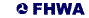United States Department of Transportation - Federal Highway Administration